## How to Get Row Number From a Vlookup in Excel

This post will guide you how to get row number from a Vlookup in Excel. How do I return a row number from a Vlookup with a formula in Excel.

Assuming that you have a list of data in range A1:B5, you can use the VLOOKUP function to lookup a value in the first column and then return the corresponding cell value in the same row. But now I do not want to get the cell value, and I want to get the row number from a VLookup in Excel. How to accomplish it.

## 1. Get Row Number From a Vlookup

If you want to return a row number from a Vlookup, you can use a formula based on the MATCH function. For example, you want to lookup a string value “excel” in range A1:A5 and return the row number where the searching value is found. Like this:

``=MATCH("excel",A2:A5,0)``

You need to type this formula into a desired cell and press Enter key to apply this formula. You would see that the row number returned.

Note: the above formula only returns a relative row number, and if you want to get a absolute row number, you can use another formula based on the ROW function and the MATCH function, like this:

``=MATCH("excel",A1:A5,0) + ROW(A1:A5)-1``

Type this formula into a blank cell and press Enter key to apply it.

## 2. Video: Get Row Number from a VLOOKUP in Excel

This video will show you how to use the MATCH function to get a relative row number and how to combine it with the ROW function to get an absolute row number from a VLOOKUP in Excel.

## 3. Related Functions

• Excel VLOOKUP function
The Excel VLOOKUP function lookup a value in the first column of the table and return the value in the same row based on index_num position.The syntax of the VLOOKUP function is as below:= VLOOKUP (lookup_value, table_array, column_index_num,[range_lookup])….
• Excel ROW function
The Excel ROW function returns the row number of a cell reference.The ROW function is a build-in function in Microsoft Excel and it is categorized as a Lookup and Reference Function.The syntax of the ROW function is as below:= ROW ([reference])….
• Excel MATCH function
The Excel MATCH function search a value in an array and returns the position of that item.The syntax of the MATCH function is as below:= MATCH  (lookup_value, lookup_array, [match_type])….

## How to Transpose Values Based on the Multiple Lookup Criteria

This post explains that how to transpose values from columns to rows based on the multiple lookup criteria in excel.

In the previous, we talked that how to transpose values from columns to rows using Paste Special Transpose, it’s just rearrange all data in a range and do not apply for any criteria.

## 1. Transpose Values Based on the Multiple Lookup Criteria

If you want to lookup the value with multiple criteria, and then transpose the last results, you can use the INDEX function with the MATCH function to create a new formula.

For example, to transpose the values in both column B and Column C based on the multiple criteria: member’s name is equal to the range B2:B10, and month’s value is equal to the range C2:C10, then extract the bonus value from the range D2:D10. you can use the following array formula:

``=INDEX(\$D\$2:\$D\$10,MATCH(1,(\$B\$2:\$B\$10=\$F4)*(\$C\$2:\$C\$10=G\$3),0))``

Let’s see how the above formula works:

=(\$B\$2:\$B\$10=\$F4)

The above formula will check if each value in the range B2:B10 is equal to the value in Cell F4, if so, return TRUE, otherwise, returns FALSE. So the above formula returns an array result like this:

``{TRUE;FALSE;FALSE;TRUE;FALSE;FALSE;TRUE;FALSE;FALSE}``

=(\$C\$2:\$C\$10=G\$3)

The above formula will check if each month value in the range C2:C10 is equal to the value in Cell G3, if so, return TRUE, otherwise, returns FALSE. So the above formula returns an array result like this:

``{TRUE;TRUE;TRUE;FALSE;FALSE;FALSE;FALSE;FALSE;FALSE}``

=(\$B\$2:\$B\$10=\$F4)*(\$C\$2:\$C\$10=G\$3)

the above formula returns an array result like this:

``{1;0;0;0;0;0;0;0;0}``

= MATCH(1,(\$B\$2:\$B\$10=\$F4)*(\$C\$2:\$C\$10=G\$3),0)

The MATCH function used the above array result(containing one and zero) to find the position of item “1”, it is actually the position of the bonus value that matched the multiple criteria.

=INDEX(\$D\$2:\$D\$10,MATCH(1,(\$B\$2:\$B\$10=\$F4)*(\$C\$2:\$C\$10=G\$3),0))

The INDEX function extracts the value based on the position result returned by the above MATCH function. So it returns “\$100”in Cell G4.

You can drag the Fill Handler in the Cell G4 to other cells to fill up the entire range G4:I6 as you need.

## 2. Video: Transpose Values Based on the Multiple Lookup Criteria in Excel

This video will show you how to use an array formula to transpose values based on multiple lookup criteria in Excel.

## 3. Related Formulas

• Reverse a List or Range
If you want to reverse a list or range, you can use a combination of the INDEX function, the COUNTA function, the ROW function or ROWS function to create a new formula. you can use the following formula:=INDEX(\$A\$2:\$A\$5,COUNTA(\$A\$2:\$A\$5)-ROWS(\$C\$2:C2)+1)…
• Lookup the Value with Multiple Criteria
If you want to lookup the value with multiple criteria in a range, you can use a combination with the INDEX function and MATCH function to create an array formula…

## 4. Related Functions

• Excel INDEX function
The Excel INDEX function returns a value from a table based on the index (row number and column number)The INDEX function is a build-in function in Microsoft Excel and it is categorized as a Lookup and Reference Function.The syntax of the INDEX function is as below:= INDEX (array, row_num,[column_num])…
• Excel MATCH function
The Excel MATCH function search a value in an array and returns the position of that item.The syntax of the MATCH function is as below:= MATCH  (lookup_value, lookup_array, [match_type])….

## How to Concatenate Text based on unique values in Another Column in Excel

This post will guide you how to concatenate text values based on unique values in another column in Excel. How do I concatenate cells based on specific criteria in Excel.

## 1. Concatenate Text Based on unique Values in Another Column

Assuming that you have a list of data in range A1:B6, in which contain product IDs and product Names. And you want to concatenate product names based on unique ID values (There are duplicated ID values in Column A), How to do it. You need to extract unique product IDs in another range, and then concatenating text values based on newly created range with a User Defined Function. Here are the steps:

Step1: you can use an Excel Array formula based on the IFERROR function, the INDEX function, the MATCH function and the COUNTIF function to extract the unique product ID values.

``=IFERROR(INDEX(\$A\$2:\$A\$6, MATCH(0,COUNTIF(\$C\$1:C1, \$A\$2:\$A\$6), 0)),"")``

Type this formula into cell C2, and press Ctrl + Shift + Enter keys on your keyboard to change it as array formula.  And then drag the AutoFill Handle down to other cells until getting blank cells.

Step2: open your excel workbook and then click on “Visual Basic” command under DEVELOPER Tab, or just press “ALT+F11” shortcut.

Step3: then the “Visual Basic Editor” window will appear.

Step4: click “Insert” ->”Module” to create a new module.

Step5: paste the below VBA code (code from here) into the code window. Then clicking “Save” button.

```Function Combinerows(CriteriaRng As Range, Criteria As Variant, _
ConcatenateRng As Range, Optional Delimeter As String = " , ") As Variant
Dim i As Long
Dim strResult As String
On Error GoTo ErrHandler
If CriteriaRng.Count <> ConcatenateRng.Count Then
Combinerows = CVErr(xlErrRef)
Exit Function
End If

For i = 1 To CriteriaRng.Count
If CriteriaRng.Cells(i).Value = Criteria Then
strResult = strResult & Delimeter & ConcatenateRng.Cells(i).Value
End If
Next i

If strResult <> "" Then
strResult = Mid(strResult, Len(Delimeter) + 1)
End If

Combinerows = strResult
Exit Function
ErrHandler:
Combinerows = CVErr(xlErrValue)
End Function```

Step6: back to the current worksheet, then type the following formula in a blank cell, and then press Enter key.

``=combinerows(A2:A6,C2,B2:B6)``

Step7: drag the AutoFill handle over other cells to  concatenate text based on unique product ID values.

## 2. Video: Concatenate Text based on unique values in Another Column

This video will show how to use a formula in combination with a User defined function with VBA code to concatenate text based on unique values in another column in Excel.

## 3. Related Functions

• Excel IFERROR function
The Excel IFERROR function returns an alternate value you specify if a formula results in an error, or returns the result of the formula.The syntax of the IFERROR function is as below:= IFERROR (value, value_if_error)…
• Excel COUNTIF function
The Excel COUNTIF function will count the number of cells in a range that meet a given criteria. This function can be used to count the different kinds of cells with number, date, text values, blank, non-blanks, or containing specific characters.etc.= COUNTIF (range, criteria)…
• Excel INDEX function
The Excel INDEX function returns a value from a table based on the index (row number and column number)The INDEX function is a build-in function in Microsoft Excel and it is categorized as a Lookup and Reference Function.The syntax of the INDEX function is as below:= INDEX (array, row_num,[column_num])…
• Excel MATCH function
The Excel MATCH function search a value in an array and returns the position of that item.The syntax of the MATCH function is as below:= MATCH  (lookup_value, lookup_array, [match_type])….

## How to Find the Largest Value in a Column and Return the Adjacent Cell Value in Excel

This post will guide you how to find the largest value in a column and then return the adjacent cell value with a formula in Excel. How do I find the max value and return the cell value from the left adjacent column in Excel.

## 1. Find the Largest Value and Return the Adjacent Cell Value

Assuming that you have a list of data in range A1:B5, and you want to find the largest value from the Sales column and then return the adjacent cell value in product column. How to do it. You can use a formula based on the VLOOKUP function and the MAX function to achieve the result. Like this:

``=VLOOKUP(MAX(A2:A5), A2:B5, 2, FALSE)``

Type this formula into a blank cell and press Enter key on your keyboard.

You should see that the adjacent cell value is extracted.

The A2:B5 is the data range that you want to use in the VLOOKUP function.  And the Number 2 is the column number that the matched value is returned, it should be the product column number.

## 2. Find the Max Value and Return Value from Left Adjacent Cell

If you want to find the max value in Sales column and return value from the left adjacent cell in your worksheet, you need to use the INDEX function to create a formula in combination with the MATCH function and the MAX function. Like this:

``=INDEX(A2:A5,MATCH(MAX(B2:B5),B2:B5,0))``

Type this formula into a blank cell and press Enter key on your keyboard.

## 3. Video: Find the Largest Value in a Column and Return the Adjacent Cell Value in Excel

This video will demonstrate how to use a formula to find the largest value in a column and return the adjacent cell value in Excel.

## 4. Related Functions

• Excel INDEX function
The Excel INDEX function returns a value from a table based on the index (row number and column number)The INDEX function is a build-in function in Microsoft Excel and it is categorized as a Lookup and Reference Function.The syntax of the INDEX function is as below:= INDEX (array, row_num,[column_num])…
• Excel MATCH  function
The Excel MATCH function search a value in an array and returns the position of that item.The MATCH function is a build-in function in Microsoft Excel and it is categorized as a Lookup and Reference Function.The syntax of the MATCH function is as below:= MATCH  (lookup_value, lookup_array, [match_type])….
• Excel MAX function
The Excel MAX function returns the largest numeric value from the numbers that you provided. Or returns the largest value in the array.= MAX(num1,[num2,…numn])…
• Excel VLOOKUP function
The Excel VLOOKUP function lookup a value in the first column of the table and return the value in the same row based on index_num position.The syntax of the VLOOKUP function is as below:= VLOOKUP (lookup_value, table_array, column_index_num,[range_lookup])….

## Convert State Names To Abbreviations

Assume that you got a task to convert the full state’s name into the abbreviations in MS Excel, and for doing this task, you might choose to do it manually, which is an acceptable way only if you don’t have any other way to do it!

But fortunately, there is a way to convert a state’s name into abbreviations, and after reading this article carefully, you would get to know about that way by which you can do this task in a few seconds.

So let’s get straight into it.

## 1. Convert State Names To Abbreviations

#### a. General Formula

The Formula to convert state names to the abbreviations in a matter of seconds is mentioned as follows:

``=VLOOKUP(States_Name,states_Data,2,0)``

#### b. Explanation of Syntax

Before getting knowledge about how to use this Formula to get the work done, we first need to understand how each syntax of the Formula contributes to making this Formula worthful:

• `VLOOKUP`: The VLOOKUP function retrieves data from a range or table row by row.
• `Parenthesis` (): The primary function of this symbol is to group the elements.

#### Let’s See How This Formula Works

A simple formula based on VLOOKUP may be used to convert complete state names to their two-letter abbreviation. The Formula in B2 in the example is:

``=VLOOKUP(A2, states_Data,2,0)``

Where “ states_Data ” refers to the designated E2:F7 range:

This means that this method is based on a database with columns for the full state name and the 2-letter abbreviation. Because we’re using VLOOKUP, we need the whole name in the first column. The table has been dubbed “states_Data” for clarity’s sake.

VLOOKUP is set to retrieve the lookup value from column A. The table array represents the designated range “ states_Data,” The column index is 2 (to extract the abbreviation from the second column). The final option, range lookup, has been set to zero (FALSE).

VLOOKUP finds the matching entry in the “states_Data” database and returns the 2-letter abbreviation.

## 2. Convert Abbreviations to State Names (Lookup In Reverse)

What if you have an abbreviation of the states and want to search up the complete state name in the sample lookup table? You’ll need to use INDEX and MATCH instead in such a situation. With a lookup value in A2, this Formula will yield the whole state name as shown in the lookup table:

``=INDEX(D2:D7,MATCH(A2,E2:E7,0))``

You may use this version to convert a 2-letter abbreviation to a complete state name if you wish to utilize the same-named range “ states_Data.”

``=INDEX(INDEX(states_Data,0,1),MATCH(A1,INDEX(states_Data,0,2),0))``

By specifying a row number of zero, we may utilize INDEX to return whole columns. This is an interesting and helpful aspect of the INDEX function: if you specify zero for the row, you receive complete column.

You may convert abbreviations to complete state names using the INDEX and MATCH methods. The INDEX and MATCH functions can help you out to retrieve the complete state names depending on the abbreviations.

## 3. Video: Convert State Names To Abbreviations

This video will show you how to convert state names to abbreviations in Excel using a formula.

## 4. Related Functions

• Excel VLOOKUP function
The Excel VLOOKUP function lookup a value in the first column of the table and return the value in the same row based on index_num position.The syntax of the VLOOKUP function is as below:= VLOOKUP (lookup_value, table_array, column_index_num,[range_lookup])….
• Excel INDEX function
The Excel INDEX function returns a value from a table based on the index (row number and column number)The INDEX function is a build-in function in Microsoft Excel and it is categorized as a Lookup and Reference Function.The syntax of the INDEX function is as below:= INDEX (array, row_num,[column_num])…
• Excel MATCH  function
The Excel MATCH function search a value in an array and returns the position of that item.The MATCH function is a build-in function in Microsoft Excel and it is categorized as a Lookup and Reference Function.The syntax of the MATCH function is as below:= MATCH  (lookup_value, lookup_array, [match_type])….

## How to Count Only Unique Values Excluding Duplicates in Excel

We enter a list of numbers or products and there are some duplicates in the list, if we want to just do count for the unique values and exclude the duplicates, how can we do? Now you can follow the below steps to solve this question by formula quickly.

Prepare a list of products and there are some duplicates among the list. See example below:

And we want to count the total product type in one year, how can we do count?

If we just use sum formula to do count, then duplicates will be included, so we need another formula to do count excludes the duplicates. See steps below.

## 1. Count Unique Values Excluding Duplicates by Formula in Excel

Step 1: In E2 which is saved the total product type number, enter the formula:

``=SUMPRODUCT(1/COUNTIF(B1:B11,B1:B11))``

Where B1:B11 is the range you want to count the unique values.

Step 2: Click Enter and get the result in E2. We can check the result is 6, and the duplicates are not included.

In above sample, we do count for unique values and get the result 6, because we have six products A\B\C\D\E\F, and if we want to only count the unique values exclude all duplicates like product A\B\C (they appeared more than one season) how can we do count? See below steps.

## 2. Count Unique Values Excluding All Duplicates by Formula in Excel

Step 1: In E2 which is saved the total product type number, enter the formula:

``=SUM(IF(FREQUENCY(MATCH(B1:B11,B1:B11,0),ROW(B1:B11)-ROW(B1)+1)=1,1))``

Where B1:B11 is the range you want to count the unique values.

Step2: Click Enter and get the result in E2. We can check the result is 3 (Product D\E\F are unique values and only appeared in one season).

## 3. Video: Count Only Unique Values Excluding Duplicates

If you want to learn how to count only unique values excluding duplicates in Excel, this video will show you a simple and effective formula that you can use in any situation.

## 4. Related Functions

• Excel SUMPRODUCT function
The Excel SUMPRODUCT function multiplies corresponding components in the given one or more arrays or ranges, and returns the sum of those products.The syntax of the SUMPRODUCT function is as below:= SUMPRODUCT (array1,[array2],…)…
• Excel COUNTIF function
The Excel COUNTIF function will count the number of cells in a range that meet a given criteria. This function can be used to count the different kinds of cells with number, date, text values, blank, non-blanks, or containing specific characters.etc.= COUNTIF (range, criteria)…
• Excel ROW function
The Excel ROW function returns the row number of a cell reference.The ROW function is a build-in function in Microsoft Excel and it is categorized as a Lookup and Reference Function.The syntax of the ROW function is as below:= ROW ([reference])….
• Excel MATCH function
The Excel MATCH function search a value in an array and returns the position of that item.The syntax of the MATCH function is as below:= MATCH  (lookup_value, lookup_array, [match_type])…
• Excel IF function
The Excel IF function perform a logical test to return one value if the condition is TRUE and return another value if the condition is FALSE. The IF function is a build-in function in Microsoft Excel and it is categorized as a Logical Function.The syntax of the IF function is as below:= IF (condition, [true_value], [false_value])….
• Excel SUM function
The Excel SUM function will adds all numbers in a range of cells and returns the sum of these values. You can add individual values, cell references or ranges in excel.The syntax of the SUM function is as below:= SUM(number1,[number2],…)…

## How To Align Duplicate Values within Two Columns in Excel

This post will guide you how to align duplicate values within two columns based on the first column in your worksheet in Excel. How do I use an formula to align two columns duplicate values in Excel.

## 1. Aligning Duplicate Values in Two Columns using Formula

Assuming that you have two columns including product names in your worksheet. and you want to find duplicate values in those two columns(Column A and Column B) and align them based on the first column in a new column(Column A and Column B). How to do it. And you can use an formula based on the `IF function`, the `ISNA function`, the `MATCH function`, and the `INDEX function`. Like below:

``=IF(ISNA(MATCH(A1,\$B\$1:\$B\$7,0)),"",INDEX(\$A\$1:\$B\$7,MATCH(A1,\$B\$1:\$B\$7,0),2))``

Type this formula into the first cell in Column C, and press Enter key on your keyboard to apply it. then drag the AutoFill handle down to other cells to apply this formula.

Note: Cell A1 is the first cell in the first column, and B1:B7 is the range of cells in the second column.

## 2. Aligning Duplicate Values in Two Columns with VBA Code

You can also align duplicate values within two columns using VBA Macro in Excel, just do the following steps:

Step1: Press “Alt” and “F11” keys at the same time to open the Visual Basic Editor.

Step2: In the Visual Basic Editor, select “Insert” from the menu bar, then choose “Module” to create a new module.

Step3: Copy and paste the below VBA code into the new module. Close the Visual Basic Editor and return to the Excel sheet.

```Sub CheckDuplicateValues_ExcelHow()
Dim sourceRange As Range, lookupRange As Range, outputRange As Range
Dim i As Long, matchIndex As Variant

' prompt the user to select the source range
On Error Resume Next ' to handle the cancel button
Set sourceRange = Application.InputBox("Select the source range:", Type:=8)
On Error GoTo 0 ' reset the error handling

If sourceRange Is Nothing Then Exit Sub ' exit if the user cancels

' prompt the user to select the lookup range
On Error Resume Next
Set lookupRange = Application.InputBox("Select the lookup range:", Type:=8)
On Error GoTo 0

If lookupRange Is Nothing Then Exit Sub

' prompt the user to select the output range
On Error Resume Next
Set outputRange = Application.InputBox("Select the output range:", Type:=8)
On Error GoTo 0

If outputRange Is Nothing Then Exit Sub

For i = 1 To sourceRange.Cells.Count
matchIndex = Application.Match(sourceRange.Cells(i), lookupRange, 0)
If IsError(matchIndex) Then
outputRange.Cells(i).Value = ""
Else
outputRange.Cells(i).Value = Application.Index(sourceRange.Resize(, 2), matchIndex, 2)
End If
Next i
End Sub```

Step4: Press “Alt” and “F8” keys at the same time to open the Macro dialog box. Select the macro ” CheckDuplicateValues_ExcelHow” from the list and click the “Run” button to execute the code.

Step5: The code will prompt you to select the source range, lookup range, and output range.

Step6: The code will run and populate the output range with the values that match between the source and lookup ranges.

You should now have the results in the output range.

## 3. Video: Aligning Duplicate Values in Two Columns

This video will show you how to align duplicate values within two columns in Excel using both a formula and a VBA code.

## 4. Related Functions

• Excel IF function
The Excel IF function perform a logical test to return one value if the condition is TRUE and return another value if the condition is FALSE. The IF function is a build-in function in Microsoft Excel and it is categorized as a Logical Function.The syntax of the IF function is as below:= IF (condition, [true_value], [false_value])….
• Excel INDEX function
The Excel INDEX function returns a value from a table based on the index (row number and column number)The INDEX function is a build-in function in Microsoft Excel and it is categorized as a Lookup and Reference Function.The syntax of the INDEX function is as below:= INDEX (array, row_num,[column_num])…
• Excel MATCH  function
The Excel MATCH function search a value in an array and returns the position of that item.The MATCH function is a build-in function in Microsoft Excel and it is categorized as a Lookup and Reference Function.The syntax of the MATCH function is as below:= MATCH  (lookup_value, lookup_array, [match_type])….
• Excel ISNA function
The Excel ISNA function used to check if a cell contains the #N/A error, if so, returns TRUE; otherwise, the ISNA function returns FALSE.The syntax of the ISNA function is as below:=ISNA(value)….

## Find Missing Numbers in a Sequence in Excel

If you’re working with data in Excel and notice that there are some missing numbers in a sequence, it can be frustrating to try and figure out where they are. Fortunately, there are a few ways to find these missing numbers quickly and easily.

This post will guide you how to find missing numbers in a sequence with a formula in Excel. How do I identify missing numbers in a consecutive series in Excel. How to find missing serial number in Excel 2013/2016.

This post will guide you through two methods to find missing numbers in a sequence in Excel: using a formula and using VBA code.

## 1. Find Missing Numbers in a Sequence in Excel

Assuming that you have a serial number list in Column B, and you want to find the missing number in this sequence list. How to achieve it.

You can use an excel array formula based on the SMALL function, the IF function, the ISNA function, the MATCH function, and the ROW function. Like this:

``=SMALL(IF(ISNA(MATCH(ROW(B\$1:B\$20),B\$1:B\$20,0)),ROW(B\$1:B\$20)),ROW(B1))``

Type this array formula into a blank cell, and then press Ctrl + Shift + Enter keys in your keyboard.

The missing numbers are listed in cells.

Or you can use another array formula based on the Small function, the IF function, the Countif function and the row function to achieve the same result. Like this:

``=SMALL(IF(COUNTIF(\$B\$1:\$B\$10,ROW(\$1:\$20))=0,ROW(\$1:\$20),""),ROW(B1))``

## 2. Find Missing Numbers in a Sequence using VBA Code in Excel

To find missing numbers in a sequence in Excel with VBA code, you can follow these steps:

Step1: Press Alt + F11 to open the Visual Basic Editor (VBE) or click Developer > Visual Basic.

Step2: In the VBE window, right-click your workbook and click Insert > Module.

Step3: In the module window, paste the following code:

```Sub FindMissingNumbers_ExcelHow()
Dim rng As Range
Dim outRng As Range
Dim i As Long
Dim n As Long
Dim found As Range

' Prompt the user to select the range of data to export
Set rng = Application.InputBox(prompt:="Select the range that contain a sequence list", Type:=8)
Set outRng = Application.InputBox(prompt:="Select one cell as output range", Type:=8)

n = Application.Min(rng) 'get the minimum number in the input range

For i = 1 To Application.Max(rng) - n + 1 '
Set found = rng.Find(n, LookIn:=xlValues, LookAt:=xlWhole)
If found Is Nothing Then
outRng.Value = n
Set outRng = outRng.Offset(1)
End If
n = n + 1
Next i

End Sub```

Step4: Press ALT + F8 on your keyboard to open the Macro dialog box. Select the FindMissingNumbers_ExcelHow macro from the list and click the Run button.

Step5: Select one range of cells that contains a sequence to find missing numbers.

Step6: Select one cell as output range to place the missing numbers.

Step7: The missing numbers in the sequence will be listed in the output range.

## 3. Video: Find Missing Numbers in a Sequence in Excel

This video will demonstrate both a formula and VBA code to help you find missing numbers in a sequence in Excel.

## 4. Related Functions

• Excel MATCH function
The Excel MATCH function search a value in an array and returns the position of that item.The syntax of the MATCH function is as below:= MATCH  (lookup_value, lookup_array, [match_type])….
• Excel SMALL function
The Excel SMALL function returns the smallest numeric value from the numbers that you provided. Or returns the smallest value in the array.The syntax of the SMALL function is as below:=SMALL(array,nth) …
• Excel ROW function
The Excel ROW function returns the row number of a cell reference.The ROW function is a build-in function in Microsoft Excel and it is categorized as a Lookup and Reference Function.The syntax of the ROW function is as below:= ROW ([reference])….
• Excel IF function
The Excel IF function perform a logical test to return one value if the condition is TRUE and return another value if the condition is FALSE. The IF function is a build-in function in Microsoft Excel and it is categorized as a Logical Function.The syntax of the IF function is as below:= IF (condition, [true_value], [false_value])….
• Excel COUNTIF function
The Excel COUNTIF function will count the number of cells in a range that meet a given criteria. This function can be used to count the different kinds of cells with number, date, text values, blank, non-blanks, or containing specific characters.etc.= COUNTIF (range, criteria)…

## How to Look Up the Lowest Value in A List by VLOOKUP/INDEX/MATCH Functions in Excel

VLOOKUP function is very useful in our daily work and we can use it to look up match value in a range, then get proper returned value (the returned value may be just adjacent to the match value). Sometimes we only want to look up the lowest value among all matched values in the list and get its adjacent value, how can we do?

This tutorial will help you to look up the lowest value in a list by VLOOKUP function and User Defined Function with VBA code. However, except VLOOKUP function, we can also use INDEX/MATCH functions together in some situations to look up the lowest value as well. Please see details below.

Precondition:

Prepare a table consists of name, score and range columns. Now we want to get the ‘Range’ of the lowest score.

## 1. Look Up the Lowest Value by VLOOKUP Function

Step1: In E2, enter the formula:

``=VLOOKUP(MIN(B2:B6),B2:C6,2,FALSE)``

As we want to find the ‘Range’ of the lowest score, we need to look up the lowest score among all score first, so we enter MIN(B2:B6) as lookup_value.

Step2: Click Enter to get returned value in C column.

Comment:

a.If there are two duplicate lowest values, VLOOKUP function will look up the first match value and return its adjacent value.

b.This method only works well when returned value is listed in the right column.

## 2. Look Up the Lowest Value using User Defined Function with VBA Code

You can also create a User Defined function with VBA Code to lookup the lowest value in a list to replace the above VLOOKUP formula. Just do the following steps:

Step1: Open your Excel workbook and press Alt + F11 to open the Visual Basic Editor.

Step2: In the Visual Basic Editor, click Insert > Module to insert a new module.

Step3: Copy the below VBA code for the custom function and paste it into the new module. Save the VBA module by clicking File > Save or by pressing Ctrl + S.

```Function MyVLOOKUP_Excelhow(rng As Range, lookup_value As Variant, column_index As Integer, exact_match As Boolean) As Variant
Dim lookup_range As Range
Dim result As Variant
Set lookup_range = rng
lookup_value = Application.WorksheetFunction.Min(lookup_range.Columns(1))
result = Application.WorksheetFunction.VLookup(lookup_value, lookup_range, column_index, exact_match)
MyVLOOKUP_Excelhow = result
End Function
```

Step4: In your Excel worksheet, enter a formula using the custom function just like you would any other built-in Excel function. Enter the following formula in Cell E2:

``=MyVLOOKUP_Excelhow(B2:C6,0,2,FALSE)``

Step5: Press Enter to calculate the result of the formula using the custom function.

## 3. Look Up the Lowest Value by INDEX/MATCH Functions

In above example, if we want to know the name of the lowest score, VLOOKUP function doesn’t work. So we use INDEX and MATCH functions combination to look up the lowest value here.

Step1: In B2, enter the formula:

``=INDEX(A2:A6,MATCH(MIN(B2:B6),B2:B6,0))``

MATCH function returns the location of cell, in this example it returns the lowest value’s row number. Then we can use INDEX function to get proper Name from A2:A6 refer to row number.

Step2: Click Enter to get returned value.

Comment:

a. If there are two duplicate lowest values, INDEX/MATCH functions will look up the first match value and return its adjacent value.

b. This method works well for that returned value lists on the both sides of match value.

## 4. Video: Look Up the Lowest Value in A List

This video will demonstrate how to use the VLOOKUP function and VBA code to look up the lowest value in a list in Excel.

## 5. Related Functions

• Excel INDEX function
The Excel INDEX function returns a value from a table based on the index (row number and column number)The INDEX function is a build-in function in Microsoft Excel and it is categorized as a Lookup and Reference Function.The syntax of the INDEX function is as below:= INDEX (array, row_num,[column_num])…
• Excel MAX function
The Excel MAX function returns the largest numeric value from the numbers that you provided. Or returns the largest value in the array.= MAX(num1,[num2,…numn])…
• Excel MIN function
The Excel MIN function returns the smallest numeric value from the numbers that you provided. Or returns the smallest value in the array.The MIN function is a build-in function in Microsoft Excel and it is categorized as a Statistical Function.The syntax of the MIN function is as below:= MIN(num1,[num2,…numn])….
• Excel VLOOKUP function
The Excel VLOOKUP function lookup a value in the first column of the table and return the value in the same row based on index_num position.The syntax of the VLOOKUP function is as below:= VLOOKUP (lookup_value, table_array, column_index_num,[range_lookup])….
• Excel MATCH  function
The Excel MATCH function search a value in an array and returns the position of that item.The MATCH function is a build-in function in Microsoft Excel and it is categorized as a Lookup and Reference Function.The syntax of the MATCH function is as below:= MATCH  (lookup_value, lookup_array, [match_type])….

## How to Lookup the Next Largest Value

This post will provide you with two different methods to lookup the next largest value in Excel: using a formula and using VBA code. While both methods achieve the same result, they differ in their implementation and can be used depending on your specific requirements.

## 1. Find the Next Largest Value Using Formula

If you want to get the next largest value in another column, you can use a combination of the INDEX function and the MATCH function to create an excel formula.

For example, if we used the VLOOKUP function to lookup the value 200, then it returns “103”, so it returns the value that is less than or equal to the lookup value. but if you want to return the next largest value in the range A2:A5, you can use the following formula:

``=INDEX(A2:A5,MATCH(200,A2:A5)+1)``

And if you want to find the next largest value in another column B2:B5, you just need to change the first argument of the INDEX function, use the range B2:B5 instead of A2:A5, the formula is as follwos:

``=INDEX(B2:B5,MATCH(200,A2:A5)+1)``

Let’s see how the above formula works:

=MATCH(200,A2:A5)+1

The MATCH function return the position of the lookup value 200 in the range A2:A5, if the MATCH function is not find the exact match, then it would return an approximate matched value(it should be less than or equalt to the lookup value) by default, so it returns 1.

To get the position of the next largest value, the position number returned by the match function need to add 1 again. so now we got the position of the next largest value in the range A2:A5. It returns 2.

=INDEX(A2:A5,MATCH(200,A2:A5)+1)

=INDEX(B2:B5,MATCH(200,A2:A5)+1)

The INDEX function returns the value based on the given position that returned by the above MATCH function.

so if you used the Range A2:A5, then the above INDEX function returns the next largest value in the range A2:A5.

And if you used the Range B2:B5, then it returns the next largest value in the range B2:B5.

You can also use the following array formula to achieve the same result.

``=INDEX(B2:B5,MATCH(TRUE,A2:A5>200,0))``

## 2. Find the Next Largest Value with VBA Code (User Defined Function)

You can also create a User defined function with VBA code to find the next largest value in a given range of cells in Excel. Just do the following steps:

Step1: Open your Excel workbook and press “Alt + F11” to open the Visual Basic Editor.

Step2: In the Editor, go to “Insert” > “Module” to create a new module.

Step3: Copy and paste the following code into the new module:

```Function FindNextLargest_ExcelHow(range1 As Range, range2 As Range, lookup_value As Double) As Variant
Dim match_index As Variant
Dim result As Variant
Dim i As Long

On Error Resume Next
match_index = WorksheetFunction.Match(lookup_value, range1, 0)
On Error GoTo 0

If IsError(match_index) Then
FindNextLargest_ExcelHow = CVErr(xlErrNA)
Else
For i = match_index + 1 To range1.Cells.Count
If range1.Cells(i).Value > lookup_value Then
result = range2.Cells(i).Value
Exit For
End If
Next i

If IsEmpty(result) Then
FindNextLargest_ExcelHow = CVErr(xlErrNA)
Else
FindNextLargest_ExcelHow = result
End If
End If
End Function```

Step4: Save the module by going to “File” > “Save” and then close the Editor.

Step5: In your worksheet, select the cell where you want to display the next largest value. Type the following formula into the formula bar:

``=FindNextLargest(A2:A5,B2:B5,200)``

Step6: this formula should return “word”, which is the next largest value in column A after 200.

## 3. Video: Find the Next Largest Value

This video will demonstrate how to lookup the next largest value using both a formula and VBA code.

## 4. Related Formulas

• Find the Relative Position in a Range or Table
If you want to know the relative row position for all rows in an Excel Range (B3:D6), you can use a excel Array formula as follows:=ROW(B3:D6)- ROW(B3) + 1. You can also use another excel array formula to get the same result as follows:=ROW(B3:D6)-ROW(INDEX(B3:D6,1,1))+1…
• Reverse a List or Range
If you want to reverse a list or range, you can use a combination of the INDEX function, the COUNTA function, the ROW function or ROWS function to create a new formula. you can use the following formula:=INDEX(\$A\$2:\$A\$5,COUNTA(\$A\$2:\$A\$5)-ROWS(\$C\$2:C2)+1)…

## 5. Related Functions

• Excel INDEX function
The Excel INDEX function returns a value from a table based on the index (row number and column number)The INDEX function is a build-in function in Microsoft Excel and it is categorized as a Lookup and Reference Function.The syntax of the INDEX function is as below:= INDEX (array, row_num,[column_num])…
• Excel MATCH function
The Excel MATCH function search a value in an array and returns the position of that item.The syntax of the MATCH function is as below:= MATCH  (lookup_value, lookup_array, [match_type])….
• Excel VLOOKUP function
The Excel VLOOKUP function lookup a value in the first column of the table and return the value in the same row based on index_num position.The syntax of the VLOOKUP function is as below:= VLOOKUP (lookup_value, table_array, column_index_num,[range_lookup])….

## How to get nth Match with One Criteria using INDEX/MATCH

This post will guide you how to get nth match based on one criteria with INDEX and MATCH in Excel. In the previous post, we used one excel formula to get the position of the nth occurrence of a value based on one criteria.

## 1. Get nth Match with One Criteria using INDEX/MATCH Formula

At this moment, we can also use it in the new formula to extract the nth match value using INDEX function. Like the below array formula:

``{=INDEX(array,SMALL(IF(vals=val,ROW(vals)-ROW(INDEX(vals,1,1))+1),nth))}``

For example, if you want to find the 2th occurrence of the member “jenny” in the range B2:B10 and extracts its relative bonus value in the range D2:D10, you can used the following array formula:

``=INDEX(D2:D10, SMALL(IF(B2:B10="jenny", ROW(B2:B10)-ROW(INDEX(B2:B10,1,1))+1),2))``

Let’s see how this formula works

= SMALL(IF(B2:B10=”jenny”, ROW(B2:B10)-ROW(INDEX(B2:B10,1,1))+1),2)

The detailed description for this formula, please continue reading: get the position of nth occurrence of a value in column

The SMALL function returns the position of the second occurrence of the string text “jenny” in the range B2:B10. If you want to get the third or nth match value in a range, just need to modify the nth in the formula as your need.

=INDEX(D2:D10, SMALL(IF(B2:B10=”jenny”, ROW(B2:B10)-ROW(INDEX(B2:B10,1,1))+1),2))

The First INDEX function returns bonus value of the second match based on the position number returned by the SMALL function. So it returns \$165.

## 2. Related Formulas

• Find nth Occurrence with Multiple Criteria Using INDEX/MATCH
If you want to find the nth occurrence with multiple criteria, you can use a combination with the INDEX function, SMALL function, nested IF function and ROW function to create a complex excel formula like this:=INDEX(Array,SMALL(IF(Range1…
• Reverse a List or Range
If you want to reverse a list or range, you can use a combination of the INDEX function, the COUNTA function, the ROW function or ROWS function to create a new formula. you can use the following formula:=INDEX(\$A\$2:\$A\$5,COUNTA(\$A\$2:\$A\$5)-ROWS(\$C\$2:C2)+1)…
• Transpose Values Based on the Multiple Lookup Criteria
If you want to lookup the value with multiple criteria, and then transpose the last results, you can use the INDEX function with the MATCH function to create a new formula.…
• Lookup the Value with Multiple Criteria
If you want to lookup the value with multiple criteria in a range, you can use a combination with the INDEX function and MATCH function to create an array formula.…
• Lookup the Next Largest Value
If you want to get the next largest value in another column, you can use a combination of the INDEX function and the MATCH function to create an excel formula..

## 3. Related Functions

• Excel INDEX function
The Excel INDEX function returns a value from a table based on the index (row number and column number)The INDEX function is a build-in function in Microsoft Excel and it is categorized as a Lookup and Reference Function.The syntax of the INDEX function is as below:= INDEX (array, row_num,[column_num])…
• Excel MATCH function
The Excel MATCH function search a value in an array and returns the position of that item.The syntax of the MATCH function is as below:= MATCH  (lookup_value, lookup_array, [match_type])….
• Excel SMALL function
The Excel SMALL function returns the smallest numeric value from the numbers that you provided. Or returns the smallest value in the array.The syntax of the SMALL function is as below:=SMALL(array,nth) …
• Excel ROW function
The Excel ROW function returns the row number of a cell reference.The ROW function is a build-in function in Microsoft Excel and it is categorized as a Lookup and Reference Function.The syntax of the ROW function is as below:= ROW ([reference])….
• Excel IF function
The Excel IF function perform a logical test to return one value if the condition is TRUE and return another value if the condition is FALSE. The IF function is a build-in function in Microsoft Excel and it is categorized as a Logical Function.The syntax of the IF function is as below:= IF (condition, [true_value], [false_value])….

## How to Find the Last or First Value in a Range Greater Than X in Excel

This post will guide you how to find the first value greater than a specified value in a range of cells in Excel. How do I find the last value greater that a given value in a row with a formula in Excel.

Assuming that you have a list of data in range B1:B6, in which contain numeric values. And you want to find the first number from the list that is greater than a given number (30). Or you wish to find the last one number from your data that is greater than a number 30. The below will show you one formula to accomplish it.

## 1. Find the Last Value Greater Than X Using Formula

If you want to identify the last value in your range B1:B6 that is greater that number 30, you can use the following array formula based on the LOOKUP function and the IF function. Like this:

``=LOOKUP(9.999999999E+307,IF(B1:B6>30,B1:B6))``

You can type the above formula into a blank cell in your worksheet, and make sure to press Ctrl +shift +Enter keys on your keyboard to change your formula as array formula.

## 2. Find the Last Value Greater Than X with VBA Code

You can also use a user-defined function (vba code) to find the last value greater than a given value in Excel. You just need to do the following steps:

Step1: Open your Excel workbook and press Alt + F11 to open the Visual Basic Editor.

Step2: In the editor, click on “Insert” and select “Module” from the drop-down menu.

Step3: In the new module, enter the following code, and save the module and return to the Excel worksheet.

```Function LastGreaterThanbyExcelhow(R As Range, Val As Variant) As Variant
Dim i As Integer
LastGreaterThanbyExcelhow = ""
For i = R.Cells.Count To 1 Step -1
If R.Cells(i).Value > Val Then
LastGreaterThanbyExcelhow = R.Cells(i).Value
Exit Function
End If
Next i
End Function```

Step4: In a blank cell, enter the formula:

``=LastGreaterThanbyExcelhow(A1:A6, 30)``

Where A1:A6 is the range of cells you want to search for the last value greater than the given value and “30” is the given value.

Step5: Press Enter to calculate the function and display the result.

## 3. Find the First Value Greater Than X Using Formula

If you want to find the first value in a range that is greater that number 30, you can use the following formula based on the INDEX function and the MATCH function to identify the first value in the range B1:B6 greater that number 30. Like this:

``=INDEX(B1:B6,MATCH(TRUE,INDEX(B1:B6>30,0),))``

You just need to type the above formula into a blank cell in your worksheet, and make sure to press Ctrl +shift +Enter keys on your keyboard to change your formula as array formula.

## 4. Find the First Value Greater Than X with VBA Code

You can also use a user-defined function in Excel to find the first value greater than a given value in a range of cells. Here is the VBA Code:

```Function FindFirstGreaterThanExcelhow(TargetValue As Double, RangeToSearch As Range) As Double
Dim cell As Range
For Each cell In RangeToSearch
If cell.Value > TargetValue Then
FindFirstGreaterThanExcelhow = cell.Value
Exit Function
End If
Next cell
FindFirstGreaterThanExcelhow = CVErr(xlErrValue)
End Function```

In a cell, enter the formula:

`` =FindFirstGreaterThanExcelhow(30, A1:A6)``

Where 30 is the value you want to search for and A1:A6 is the range of cells to search.

Press Enter to execute the formula and the function will return the first value greater than the target value in the specified range.

## 5. Video: Find the Last or First Value in a Range Greater Than X in Excel

This video will demonstrate how to find the last or first value in a range greater than X in Excel using a formula or a User Defined Function (UDF).

## 6. Related Functions

• Excel LOOKUP function
The Excel LOOKUP function will search a value in a vector or array.The syntax of the LOOKUP function is as below:= LOOKUP (lookup_value, lookup_vector, [result_vector])…
• Excel IF function
The Excel IF function perform a logical test to return one value if the condition is TRUE and return another value if the condition is FALSE. The IF function is a build-in function in Microsoft Excel and it is categorized as a Logical Function.The syntax of the IF function is as below:= IF (condition, [true_value], [false_value])….
• Excel INDEX function
The Excel INDEX function returns a value from a table based on the index (row number and column number)The INDEX function is a build-in function in Microsoft Excel and it is categorized as a Lookup and Reference Function.The syntax of the INDEX function is as below:= INDEX (array, row_num,[column_num])…
• Excel MATCH  function
The Excel MATCH function search a value in an array and returns the position of that item.The MATCH function is a build-in function in Microsoft Excel and it is categorized as a Lookup and Reference Function.The syntax of the MATCH function is as below:= MATCH  (lookup_value, lookup_array, [match_type])….

## How to Extract the Column Header of the Largest Value in a Row in Excel

This post will guide you how to find the largest value in a row and return column header using VBA code or a formula in Excel. How to retrieve the column header of the largest value in a row with a formula in Excel. How to return the column title of the max value in the row in Excel.

The first method involves using a VBA user-defined function to create a custom formula that can be used to find the largest value in a given row and return the header of the column where that value is located.

The second method involves using a combination of built-in Excel functions to create a formula that can achieve the same result.

In this post, we will explore both methods and provide step-by-step instructions on how to implement them.

## 1. Video: Return the Column Header of the Largest Value in a Row

This video tutorial provides step-by-step instructions on how to return the column header of the largest value in a row in Excel using VBA code, a user-defined function, and a formula.

## 2. Return the Column Header of the Largest Value in a Row using Formula

Assuming that you have a list of data in range A1:D4, in which contain sales data for each month. And you want to find the highest value for each product and return the Month column header in your worksheet. How to do it. You can use an Excel formula based on the INDEX function, the MATCH function and the MAX function. Like this:

``=INDEX(\$B\$1:\$D\$1,MATCH(MAX(B2:D2),B2:D2,0))``

You need to type this formula into cell E2 and press Enter key on your keyboard, and then copying this formula from cell E2 to range E3:E4, you would notice that you will get the column header for in every row.

Let’s see how this formula works:

The INDEX function will return a reference of the cell at the intersection of a specific row and column in range B1:D1.

The MAX function will return the maximum number from a given range B2:D2. And pass the returned result into the MATCH function as its argument.

The MATCH function will return the relative position of the maximum value in the given range.

## 3. Return the Column Header of the Largest Value in a Row with User-defined Function

You can also create a user-defined function in VBA to extract the column header of the latest value in a row in Microsoft Excel. just do the following steps:

Step1: Open your Excel workbook and press Alt + F11 to open the VBA editor.

Step2: Insert a new module by right-clicking on the project and selecting “Insert > Module“.

Step3: Copy and paste the code above into the module. Save the module and return to your Excel sheet.

```Function GetLargestValueColumnHeaderbyExcelHow(rowRange As Range) As String
Dim maxVal As Double
Dim maxCol As Integer
Dim currentCell As Range

maxVal = -1E+20 'initialize max value as a very small value

For Each currentCell In rowRange 'loop through each cell in the row range
If currentCell.Value > maxVal Then 'check if current cell value is greater than max value
maxVal = currentCell.Value 'update max value if necessary
maxCol = currentCell.Column 'update max column if necessary
End If
Next currentCell

End Function```

Step4: In a cell where you want to display the column header of the largest value in a row, enter the following formula, then press Enter to display the column header of the largest value in the selected row.

`` =GetLargestValueColumnHeader(B2:D2)``

Where B2:D2 is the range of cells representing the row you want to check.

Step5: click on the AutoFill Handler down to cell E2,E3 to apply this formula.

## 4. Conclusion

whether you prefer using VBA code or a formula, there are multiple ways to return the column header of the largest value in a row in Excel. With the help of a user-defined function, built-in functions such as VLOOKUP or INDEX/MATCH, or a combination of both, you can quickly and easily extract the information you need from your worksheet.

## 5. Related Functions

• Excel INDEX function
The Excel INDEX function returns a value from a table based on the index (row number and column number)The INDEX function is a build-in function in Microsoft Excel and it is categorized as a Lookup and Reference Function.The syntax of the INDEX function is as below:= INDEX (array, row_num,[column_num])…
• Excel MATCH  function
The Excel MATCH function search a value in an array and returns the position of that item.The MATCH function is a build-in function in Microsoft Excel and it is categorized as a Lookup and Reference Function.The syntax of the MATCH function is as below:= MATCH  (lookup_value, lookup_array, [match_type])….
• Excel MAX function
The Excel MAX function returns the largest numeric value from the numbers that you provided. Or returns the largest value in the array.= MAX(num1,[num2,…numn])…

## Match Single Criteria in Excel

No repeat statistic based on a single condition is used wildly in daily work, for example, count type of products, the duplicate types are not encountered. This article describes the way of no repeats statistics for data in a single row or a single column using an array formula.

The example below shows the operation on a one-dimensional array with no repeat statistics.

Buy some gifts to reward the top defect submitters for their contributions to the company. The core is to count how many submitters appear on this list with repeated submitters ignored.

## MATCH Function

Build a formula with EXCEL COUNT and MATCH functions:

``=COUNT(1/(MATCH(C2:C7,C:C,0)=ROW(C2:C7)))``

It is an array formula, so press Shift+Control+Enter to run it.

In this formula:

• The “Help Column” shows the logical values returned by the MATCH function.
• The MATCH function determines whether the submitter appears in the list for the first time. It returns logical `TRUE `if it detects that the submitter does not appear in the range from the first cell to the cell above it, or logical `FALSE `if the submitter already appears in the searched field.

Use “`1`” divided by the logical value array to get a new array `{1;1;#DIV/0!;1;1;#DIV/0!}`.

The COUNT function counts the numbers with errors ignored.

## COUNTIF Function

We can also use EXCEL SUM and COUNTIF functions to count without repeats.

Build a formula with EXCEL SUM and COUNTIF functions：

``=SUM(1/COUNTIF(C2:C7,C2:C7))``

It is still an array formula, press Shift+Control+Enter to run it.

In this formula:

• COUNTIF(A:A,A:A) is a classic usage in statistics to count the number of occurrences of each value in column A.
• COUNTIF (C2:C7,C2:C7) returns an array that stores the number of occurrences of each submitter in the list. The array is {3;1;3;1;1;3}.
• Use “`1`” divided by the array returned by COUNTIF function to get a new array `{1/3;1;1/3;1;1;1/3}`.
• The SUM function adds up the numbers in the array. For duplicate values, for example, Andy in the example, `1/3+1/3+1/3=1`, so there are no duplicates in the count, which completes the statistics without duplicates.

## Excel Array Operation

We have all heard of arrays. Depending on the dimensionality, they are divided into one-dimensional arrays and two-dimensional arrays. Depending on the data type, they can also be divided into numeric arrays, logical arrays, and so on. The concatenation and operation of different arrays is an important part of excel formulas. Many people are still confused about the arithmetic operations of arrays. This article will introduce the basic operations between arrays, such as addition and multiplication.

Direct operations are performed on arrays by using operators instead of using functions. Since the components of arrays include numeric, textual, logical, and error values, arrays inherit the arithmetic properties of all types of data (except error values). Numeric and logical arrays can perform regular arithmetic operations such as addition, subtraction, multiplication, and division, while text arrays can perform concatenation operations.

## Direct Operation Between Arrays and Values

Users can perform an arithmetic operation between an array and a number. The operation returns an array in the same dimension. This array contains the same number of elements. For example, if an `M*1` array and a number are added, the new array is also an `M*1 `array.

See the example below. For vertical array, elements are separated by `semicolon`, and for horizontal array, elements are separated by `comma`.

Array: {1;2;3}

Value: 4

Operation: {1;2;3}+4

Result: {5;6;7}

The gif shows the operation between a vertical array` {1;2;3}` and a number` {4}`. The new array is also a vertical array contains three numbers `{5;6;7}`.

In some versions of excel, the area where the new array is to be saved should be selected in advance, then enter the operation in the first cell, and after entering the operation, press Shift+Control+Enter to return to the new array. However, in some versions, such as office 365, the user can enter the operation directly in `E2 `and then press Enter and the new array will be saved correctly in `E2: E4`.

The formula operation process is shown in the figure:

## Direct Operations Between Two One-dimensional Arrays with Same Direction

For one-dimensional array, based on the directions we can divide array into one-dimensional vertical array and one-dimensional horizontal array.

When two one-dimensional arrays are operated directly, the elements in the same position will be operated correspondingly, and a new array is generated after the operation, which has the same direction and the same number of elements as the original array.

See the example below.

Array1: {1;2;3}

Array2: {4;5;6}

Operation: {1;2;3}+{4;5;6}

Result: {5;6;9}

The formula operation process is shown in the figure:

Here we should note that two one-dimensional arrays should have the same number of elements, otherwise some of the returned values will have an error `#N/A`.

See the example below.

Array1: {1;2;3}

Array2: {4;5;6;7}

Operation: {1;2;3}+{4;5;6;7}

Result: {5;6;9;#N/A}

Exceeded returns error `#N/A`. In this example, there is no value in `array 1` corresponding to the fourth number` {7} `in `array 2`.

In addition to basic addition, multiplication, etc., arrays can be combined into a new array, an application that is widely used in everyday work when searching for something based on multiple conditions. The following example will show you the application in working life.

Example: Query the effort based on two conditions milestone and project.

In `F3 `enter the formula :

``=INDEX(C2:C13,MATCH(F1&F2,A2:A13&B2:B13,0)).``

In this example, the two conditions are “`Milestone: 3`” and “`Project: Project B`”, these two conditions are saved in columns `A2:A13` and` B2:B13`. We use “`&`” to concentrate the two texts into one text and the two arrays into one new array. Then the formula uses the MATCH function to search for the location of the set “`F1&F2`” in the new array “`A2:A13&B2:B13`”, and the INDEX function returns the effort corresponding to “`F1&F2`” in `C2:C13`.

The arrays are as follows:

``=INDEX({1200;1000;1100;1500;1200;1300;1400;1300;1000;1100;1500;1400},MATCH("3Project B",{"1Project A";"1Project B";"1Project C";"2Project A";"2Project B";"2Project C";"3Project A";"3Project B";"3Project C";"4Project A";"4Project B";"4Project C"},0))``

## Operation Between Two One-dimensional Arrays with Different Directions

`Array1 `is a vertically oriented array, it has one column but `M` rows, so it is an` M*1 `array with elements listed in each of the `M` rows; `Array2 `is a horizontally oriented array, it has `one `row but `N` columns, so it is a `1*N` array with elements listed in each of the `N` columns.

The vertical array `M*1` and the horizontal array` 1*N `are operated directly as follows: each element of `array 1` is operated separately from each element of `array 2`, returning a two-dimensional array of `M*N`.

See the example below.

Array1: {1,2,3}

Array2: {4;5;6}

Operation: {1,2,3}+{4;5;6}

Result: {5,6,7;6,7,8;7,8,9}

The formula operation process is shown in the figure:

## Operation Between One-dimensional Array and Two-dimensional Array

If a one-dimensional array and a two-dimensional array have the same dimensions in the same direction, for example the two-dimensional array is `M*N`, the one dimensional array is` M*1` or `1*N`, then they can be operated directly as follows: elements inside each array are operated correspondingly to form a new two-dimensional array of `M*N`.

See the example below.

Array1: {1;2;3}

Array2: {1,4;2,5;3,6}

Operation: {1;2;3}*{1,4;2,5;3,6}

Result: {1,4;4,10;9,18}

The formula operation process is shown in the figure:

If the dimensions of the one-dimensional array and the two-dimensional array are different, the result will contain the error value `#N/A`.

Array1: {1;2;3}

Array2: {1,4;2,5;3,6;1,1}

Operation: {1;2;3}*{1,4;2,5;3,6;1,1}

Result: {1,4;4,10;9,18;#N/A,#N/A}

## Operation Between Two-dimensional Arrays

Two two-dimensional arrays with the same dimension can be operated directly on two elements at the same position and return a new two-dimensional array with the same dimension. For example, operating two arrays `M*N` will form a new array `M*N`.

See the example below.

Array1: {1,4;2,5;3,6}

Array2: {1,4;2,5;3,6}

Operation: {1,4;2,5;3,6}*{1,4;2,5;3,6}

Result: {1,16;4,25;9,36}

The formula operation process is shown in the figure:

If the sizes of the two two-dimensional arrays involved in the operation do not match, the resulting array takes the largest column and row of the two arrays as the new array dimensions, but the part of the array that exceeds the smaller dimension generates the error value `#N/A`.

## Break ties with helper COUNTIF and column

Suppose you got a task to adjust the values that contain the ties; what would be your first attempt to break the ties of the given value? If you are wondering about doing this task manually, let me add that you will end up exhausted, and there is a probability that you will not complete this task on time. Moreover, besides consuming a lot of time and energy, it also seems to be a cumbersome task to break the ties in the values manually.

But don’t worry about it because you have just landed on the right article, where you will get concise information to break the ties in just a matter of seconds.

So without any further ado, let’s get started.

## General formulation

The formula for breaking ties in values using column and COUNTIF is as follows.

`= (COUNTIF(range,A1)-1)*adjustment + A1`

## Explanation of Syntax

You must be aware of the syntax used in the above formula to use it to complete your task.

CountIF: In Excel, the COUNTIF function counts the number of cells that fulfill a specific condition or criteria. Learning how to utilize the COUNTIF Excel function is helpful because we frequently want to know how many objects in a huge collection are alike in a specific manner. The COUNTIF function is commonly used as a fast fix.

• The plus operator (+): This operator is used to add the values.
• Minus Operator (-): Use this symbol to subtract any two numbers.
• Multiplication (*): In this symbol, any two values or numbers will be multiplied.
• The comma symbol (,): This symbol is a separator that aids in the separation of a list of values.
• Parenthesis (): This symbol’s primary function is to group the elements.

## Summary

To break ties, use a helper column and the COUNTIF function to alter values so that they don’t have duplicates and don’t result in ties. The formula in D5 in the example is:

`= (COUNTIF(\$B\$3: B3, B3)-1)*0.01 +B3`

When you employ SMALL, LARGE, or RANK functions to rank highest and lowest values, you may encounter ties because the data contains duplicates. In this situation, one method for breaking ties is to create a helper column with altered values, then rank those values instead of the originals.

The reasoning used to alter values in this example is random – the first duplicate value will “win,” but you may modify the formula to employ appropriate logic for your specific scenario and use case.

## Explanation

This formula relies on the COUNTIF function and an increasing range to count occurrences of values. COUNTIFS uses the expanding reference to deliver a running count of occurrences rather than a total count for each value:

`=COUNTIF(\$B\$3: B3, B3)`
`= (COUNTIF(\$B\$3: B3, B3)-1)*0.01`

The result is then multiplied by 0.01 after being removed by 1 (which makes the count of all non-duplicate values zero). This is the “adjustment,” It is purposefully minimal to not significantly influence the original value.

“excel” and “ppt” both have the same sales of 500 in the scenario. Because excel is first in the list, the running count of 500 equals 1 and is canceled out by removing 1; thus, the estimate in the helper column remains unchanged:

However, the running count of 500 for ppt product is 2, therefore the estimate is adjusted:

`=B7+0.01`

Finally, instead of the original values in columns G and H, the corrected values are utilized for ranking. The formula in H3.

`=LARGE(\$C\$3:\$C\$8,F3)`

G3’s formula is as follows:

`=INDEX(\$A\$3:\$A\$8,MATCH(H3,\$C\$3:\$C\$8,0))`

## Column of temporary assistance

Suppose you don’t want to use a helper column in the final solution. In that case, you can use it temporarily to get calculated values, then use Paste Special to convert values into the original cell range.

Then you can delete the helper column.

### Related Functions

• Excel INDEX function
The Excel INDEX function returns a value from a table based on the index (row number and column number)The INDEX function is a build-in function in Microsoft Excel and it is categorized as a Lookup and Reference Function.The syntax of the INDEX function is as below:= INDEX (array, row_num,[column_num])…
• Excel MATCH  function
The Excel MATCH function search a value in an array and returns the position of that item.The MATCH function is a build-in function in Microsoft Excel and it is categorized as a Lookup and Reference Function.The syntax of the MATCH function is as below:= MATCH  (lookup_value, lookup_array, [match_type])….
• Excel COUNTIF function
The Excel COUNTIF function will count the number of cells in a range that meet a given criteria. This function can be used to count the different kinds of cells with number, date, text values, blank, non-blanks, or containing specific characters.etc.= COUNTIF (range, criteria)…
• Excel LARGE function
The Excel LARGE function returns the largest numeric value from the numbers that you provided. Or returns the largest value in the array. The syntax of the LARGE function is as below:= LARGE (array,nth)…

## Find the Closest Data to the Data Provided in Excel

In our daily work, we may encounter such an issue that to find the closest value to a certain value. In fact, Excel internal functions can help us solve this problem. In today’s article, we will show you how to find the student whose score value is closet to the score provided with help of Excel INDEX/MATCH/MIN/ABS functions.

Look at the following example, we want to know who has a score closest to score “150” or “100”.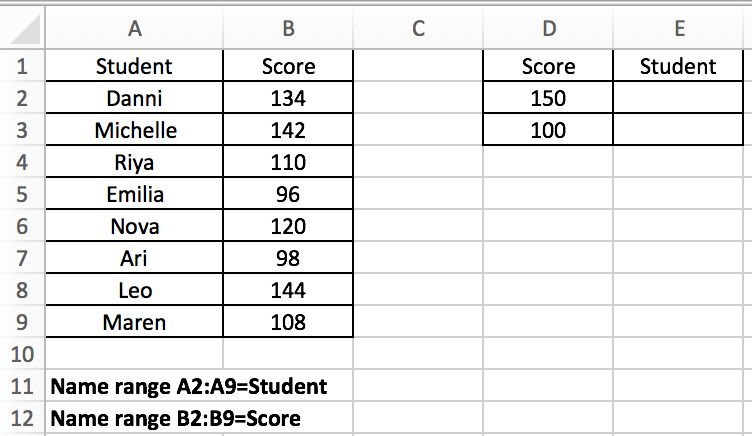Expect Result: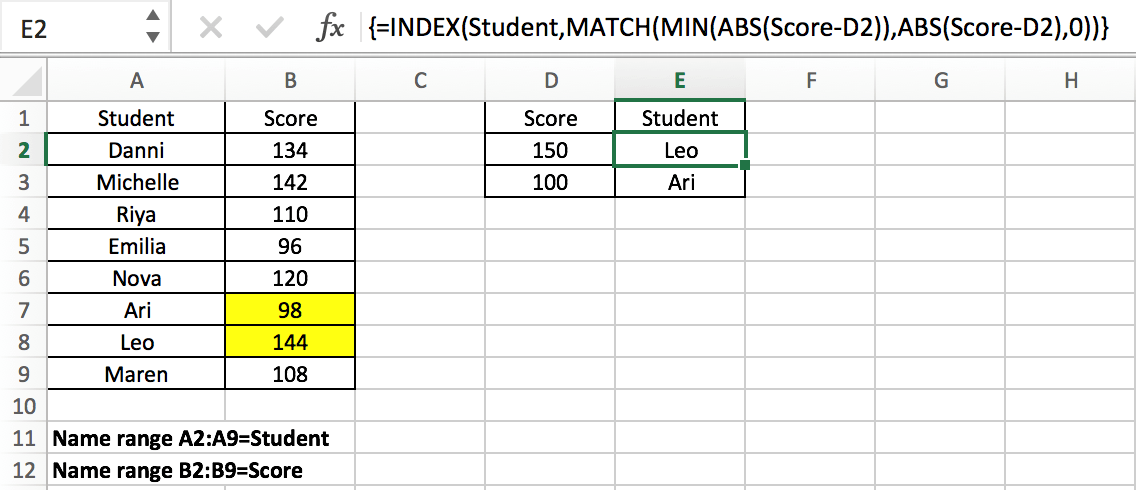## GENERAL FORMULA

The general formula for this case is

`=INDEX(Data,MATCH(MIN(ABS(Data-ProvideValue)),ABS(Data-ProvideValue),0))`

In the general formula, you can replace Data and ProvideData with your own range or data reference. This is an array formula and we need to press “control + shift + enter” after entering the formula.

In the above example, the formula is

`=INDEX(Student,MATCH(MIN(ABS(Score-D2)),ABS(Score-D2),0)).`

In the formula, Data is “Student” list (A2:A9, named “Student”); there are two provided values, 150 in D2 and 100 in D3; In this example, we want to find the closest score to the provided score (in D2 and D3) from the “Scores” list and retrieve the matching student name from the “Students” list that will be returned by the formula and filled in correctly in E2 and E3. Enter above formula in C8, then copy down the formula, the matching students are filled in properly.

Notes:

If we want to only obtain the score value instead of the student, we can just change the lookup array from Student to Score in this formula.

`=INDEX(Score,MATCH(MIN(ABS(Score-D2)),ABS(Score-D2),0))`

## EXPLANATION

For the formula, the core is the usage of Excel INDEX and MATCH functions combination. Before we can explain this formula, we need to know these two functions.

MATCH is an Excel function for locating the position of a query value in a row, column, or table. INDEX is used to return the value at a certain position. As you can see, the MATCH function can provide a relative position of a value within a range, and INDEX can provide a suitable value based on the position provided, so typically, the MATCH and INDEX functions are used together to retrieve a value at a matching position.

### Syntax:

```=MATCH(lookup_value, lookup_array, [match_type]) (match type 0=exact match)

=INDEX(array, row_num, [column_num])```

In this example, using E2 as an example, we want to return the name of the student whose score retrieved from the student list is closest to the score 150 provided in D2, so for INDEX function in this formula, the array is the named range “Student” (A2:A9). We expand this array in the formula bar, the array is generated:

`{"Danni";"Michelle";"Riya";"Emilia";"Nova";"Ari";"Leo";"Maren"}`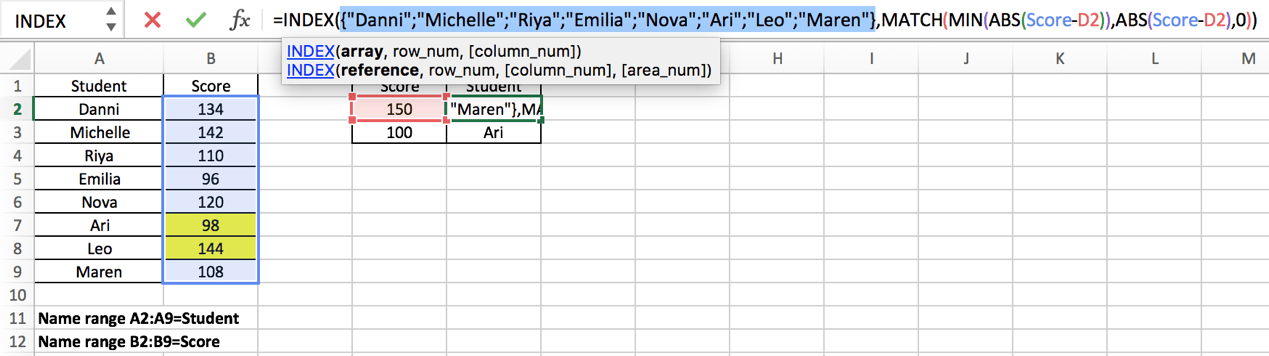In this INDEX function, the parameter “row_number” is obtained by executing another Excel MATCH function. MATCH function will delivery its result to INDEX as row number. The hard work in this case is to find the closest score in Score list to the data provided in D column and obtain the relative position of this score in Score list. To resolve this issue, we use Excel ABS and MIN functions in MATCH expression to obtain the minimum difference between the data and the data provided, and with their help, MATCH function can return the position of the closest score. To let you know how it works step by step, we will explain the expression from inside to outside as the result of the internal function will be delivered to the external one.

First, let’s get to know what the role ABS and MIN functions play in MATCH function.

ABS Function is used to return the absolute value of an integer.

Syntax:

`=ABS(value)`

MIN Function is used to return the smallest value in supplied data

Syntax:

`=MIN(number1, [number2], ...)`

In this example, MIN(ABS(Score-D2)) is the lookup value of the MATCH function. ABS(Score-D2) is the lookup array. Match type is 0, so the MATCH function returns an exact match.

Notes:

We all know that the smaller the difference between two values, the closer the two numbers are to each other. ABS(Score-D2) provides an array that save the differences between each value in “Score” and the value provided in D2, the differences may be negative or positive, but ABS function will convert the negative ones to positive numbers, so this function returns an array only contains positive numbers; And MIN(ABS(Score-D2)) provides the smallest difference among all differences.

For ABS(Score-D2), since the values saved in “Score” are vertically aligned, “Score” is a vertical array.; so (Score-D2) is also a vertical array. This step is done to get the difference between the two values.

Expand Score and D2 in the formula bar:

Score: {134;142;110;96;120;98;144;108}

D2:150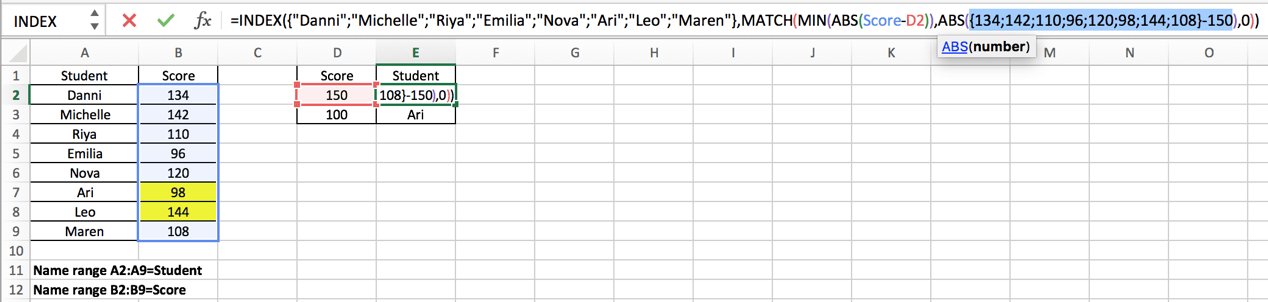Calculate (Score-D2) in Excel formula bar and get below array:

`{-16;-8;-40;-54;-30;-52;-6;-42}`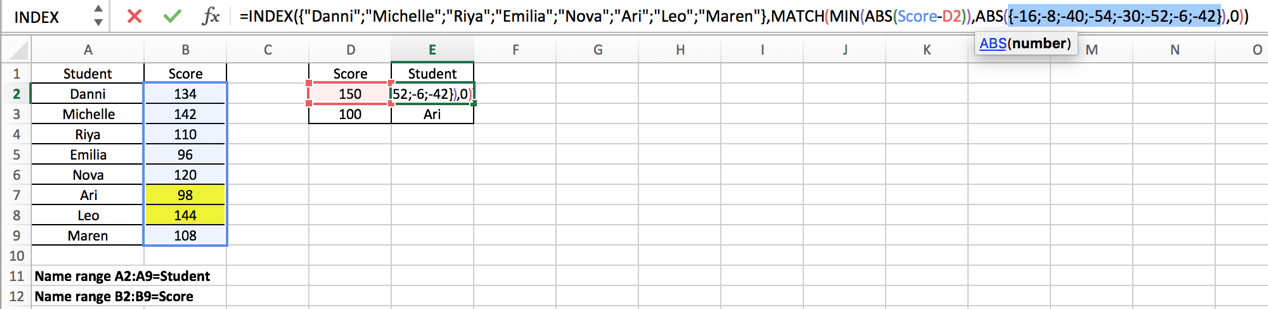Use Excel ABS function to convert all negative numbers to positive numbers.

{16;8;40;54;30;52;6;42} – This array is the lookup array for MATCH function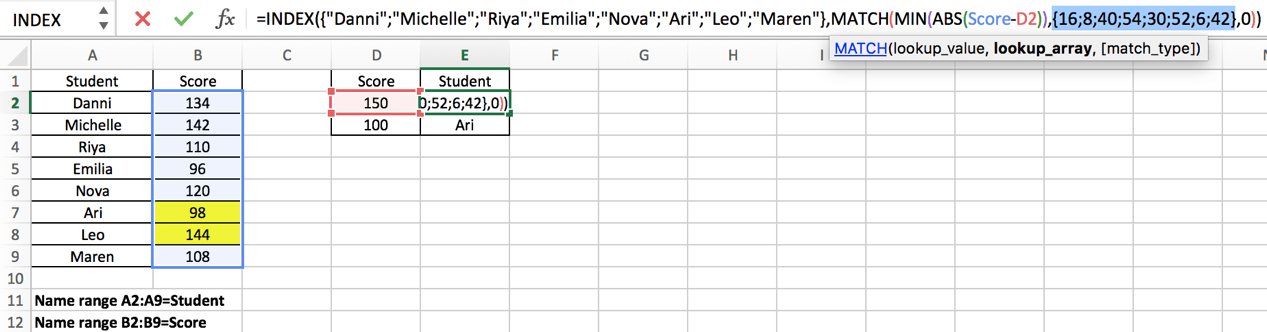For MIN(ABS(Score-D2)), the result of ABS function (numbers in the array above) is also delivered to MIN function:

### =MIN({16;8;40;54;30;52;6;42})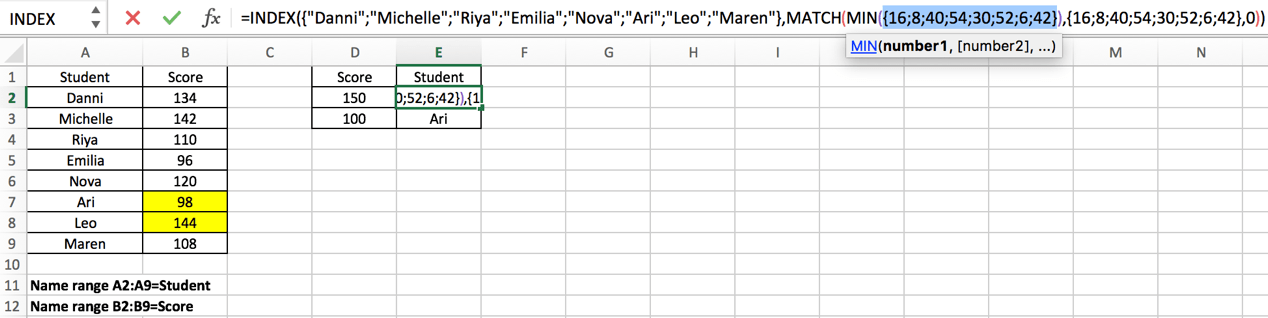The Excel MIN function will extract the smallest value in the array.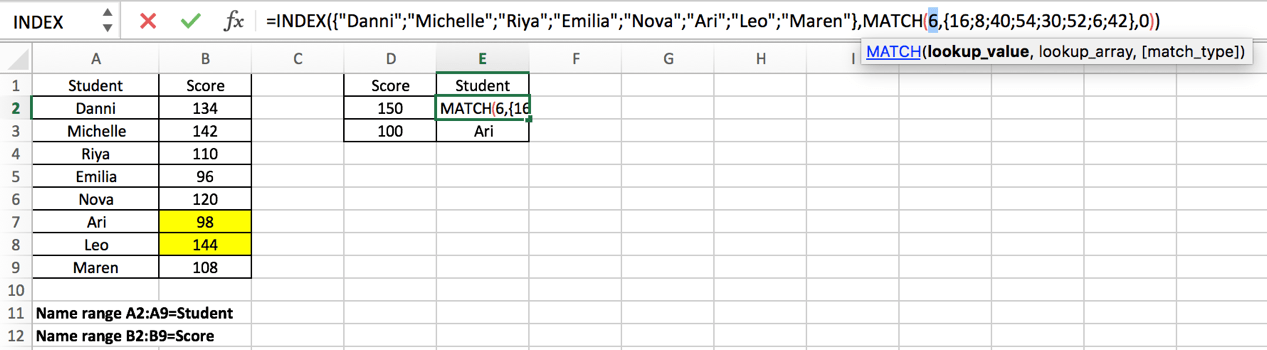Now, for MATCH function, the lookup value and lookup array are obtained after calculating ABS and MIN expressions.

### =MATCH(6,{16;8;40;54;30;52;6;42},0)

As mentioned above, Excel MATCH function returns the position of a certain value, so in this case, MATCH function returns the row number of value “6” in array {16;8;40;54;30;52;6;42}. Obviously, relative to this array, 6 is in row 7.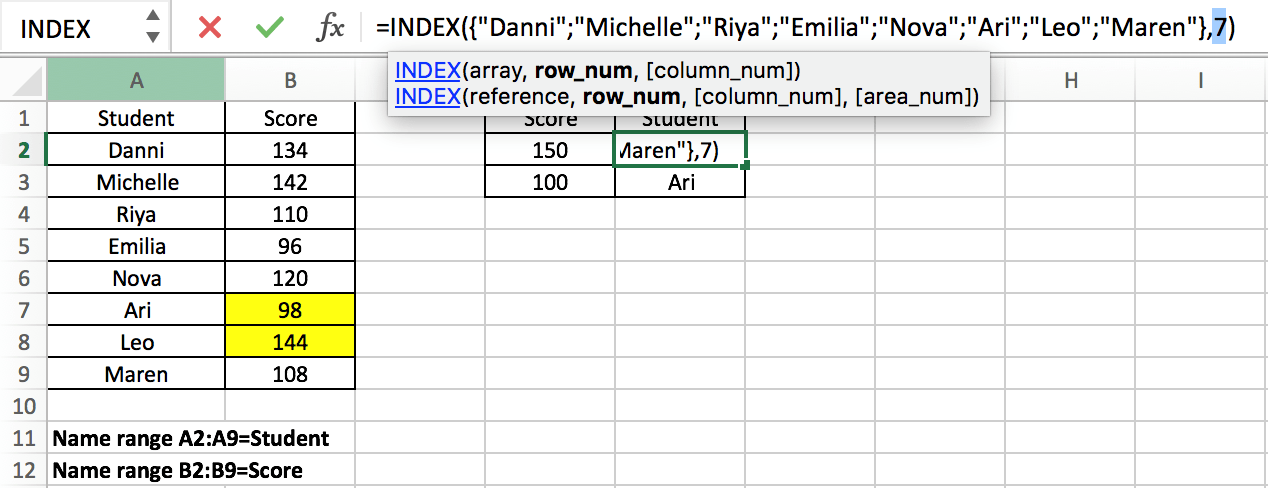Now we come to the outermost INDEX function：=INDEX({“Danni”;”Michelle”;”Riya”;”Emilia”;”Nova”;”Ari”;”Leo”;”Maren”},7)

In this formula, MATCH function delivered row number 7 to INDEX function, INDEX function returns a value in an array based on a provided row number. In this array which consists of student names, the seventh name is “Leo”, so this is the final result for this formula. After entering “Ctrl+Shift+Enter”, “Leo” is displayed in cell E2.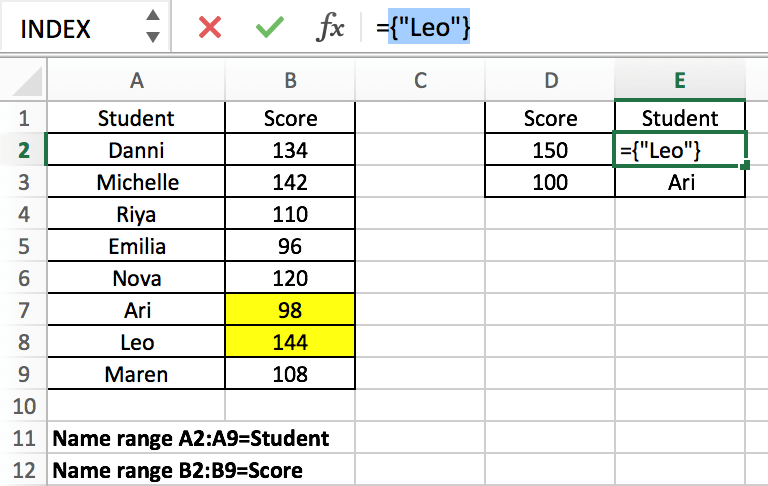Copied down the formula to E2, we can obtain “Ari” in the same way.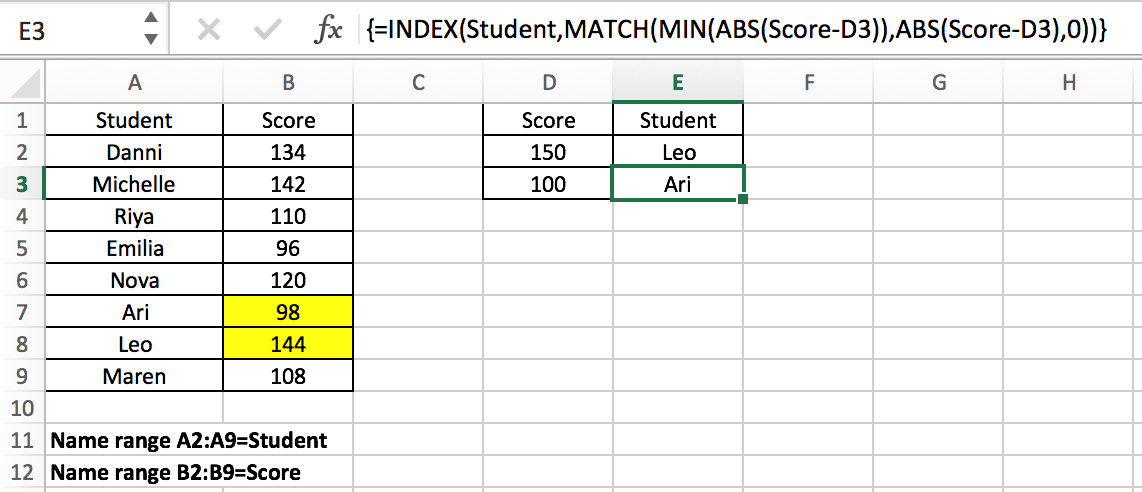This article not only introduces the joint application of INDEX and MATCH, but also the use of MIN and ABS functions, including how to apply the combination of MIN and ABS functions to find the minimum difference between values. Readers can design their own formulas according to the actual situation.

### Related Functions

• Excel INDEX function
The Excel INDEX function returns a value from a table based on the index (row number and column number)The INDEX function is a build-in function in Microsoft Excel and it is categorized as a Lookup and Reference Function.The syntax of the INDEX function is as below:= INDEX (array, row_num,[column_num])…
• Excel MATCH  function
The Excel MATCH function search a value in an array and returns the position of that item.The MATCH function is a build-in function in Microsoft Excel and it is categorized as a Lookup and Reference Function.The syntax of the MATCH function is as below:= MATCH  (lookup_value, lookup_array, [match_type])….
• Excel MIN function
The Excel MIN function returns the smallest numeric value from the numbers that you provided. Or returns the smallest value in the array.The MIN function is a build-in function in Microsoft Excel and it is categorized as a Statistical Function.The syntax of the MIN function is as below:= MIN(num1,[num2,…numn])….
• Excel ABS Function
The Excel ABS function returns the absolute value of a number.The ABS function is a build-in function in Microsoft Excel and it is categorized as a Math and Trigonometry Function.The syntax of the ABS function is as below:=ABS (number)…

## Abbreviate Names Or Words in Excel

As an MS Excel user, you might have come across a task where you need to abbreviate different names or words, and there are also possibilities that you might have done this task manually by assuming that there isn’t any other way to do this task, but you assumed wrong because fortunately there is a way which would let you abbreviate names and words in a matter of seconds unlike doing it manually which consumes a lot of time and leaves you with no satisfying results.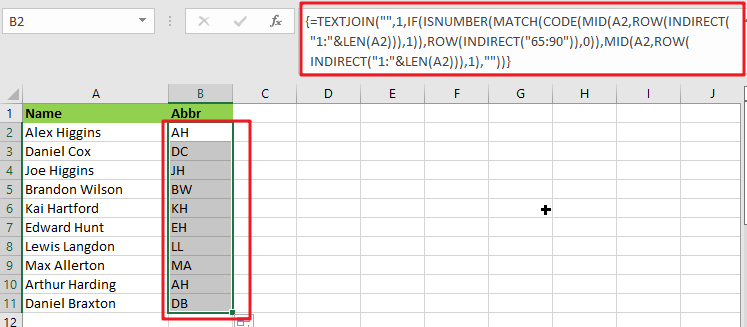So for exploring that way/method, let’s dive into the article.

## General Formula:

The array formula to abbreviate different names and words in few seconds is mentionedas follows:

`{=TEXTJOIN("",1,IF(ISNUMBER(MATCH(CODE(MID(A2,ROW(INDIRECT("1:"&LEN(A2))),1)),ROW(INDIRECT("65:90")),0)),MID(A2,ROW(INDIRECT("1:"&LEN(A2))),1),""))}`## Syntax Explanation:

To understand the working of the above formula, we first need to know about each syntax and how they contribute to abbreviating names and words in seconds.

• `TEXTJOIN`: The TEXTJOIN function in Microsoft Excel allows you to join two or more strings together, with each value separated by a delimiter. The TEXTJOIN function is an Excel built-in function classified as a String/Text Function.
• `IF`: The IF function is one of Excel’s most used functions, allowing you to create logical comparisons between a number and what you anticipate.
• `ISNUMBER`: When a cell contains a number, the ISNUMBER function returns TRUE; otherwise, it returns FALSE. ISNUMBER can be used to verify that a cell contains a numeric value or that the output of another function is a number.
• `MATCH`: The MATCH is a function used to find a lookup value in a row, column, or table in MS Excel. MATCH allows for approximate and accurate matching and wildcards (*?) for partial matches.
• `CODE`: The CODE function in Excel returns a numeric code for a specified character.
• INDIRECT: The INDIRECT function returns a range reference. This function may generate a reference that will not change if a row or column is added to the worksheet.

## Let’s See How This Formula Works:

To abbreviate capital letters text, use this array formula based on the TEXTJOIN function, new in Office 365 and Excel 2019. You may use this method to generate initials from names or acronyms. Because only capital letters can survive this algorithm, the original text must contain capitalized terms. If necessary, you can capitalize words using the PROPER function.

The formula in B2 in the case provided is:

`=TEXTJOIN("",1,IF(ISNUMBER(MATCH(CODE(MID(A2,ROW(INDIRECT("1:"&LEN(A2))),1)),ROW(INDIRECT("65:90")),0)),MID(A2,ROW(INDIRECT("1:"&LEN(A2))),1),""))`

The MID function is used to turn the string into an array of individual letters from the inside out:

`=MID(A2,ROW(INDIRECT("1:"&LEN(A2))),1)`

In this formula section, the operators MID, ROW, INDIRECT, and LEN transform a string into an array of letters.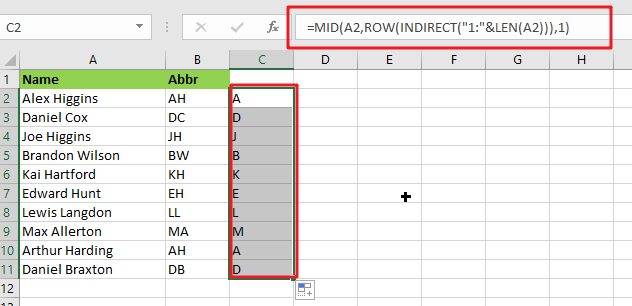MID delivers an array of all characters in the text.

`=CODE(MID(A2,ROW(INDIRECT("1:"&LEN(A2))),1))`

This above array is sent to the CODE function, which returns an array of numeric ASCII codes, one for each letter.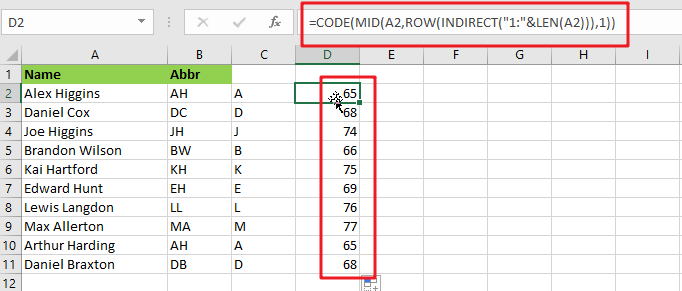`=ROW(INDIRECT("65:90")`

ROW and INDIRECT are used to generate another numeric array:

`{65;66;67;68;69;70;71;72;73;74;75;76;77;78;79;80;81;82;83;84;85;86;87;88;89;90}`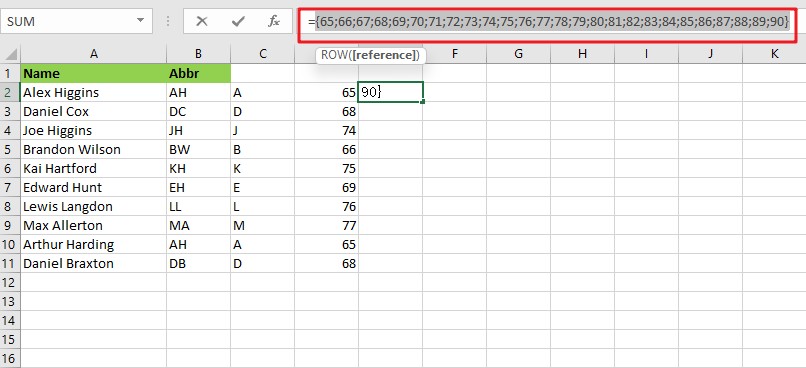Note: The digits 65 to 90 correspond to the ASCII codes for all capital letters from A to Z. This array is used as the lookup array in the MATCH function, and the original array of ASCII codes is given as the lookup value.

`{=MATCH(CODE(MID(A2,ROW(INDIRECT("1:"&LEN(A2))),1)),ROW(INDIRECT("65:90")),0)}`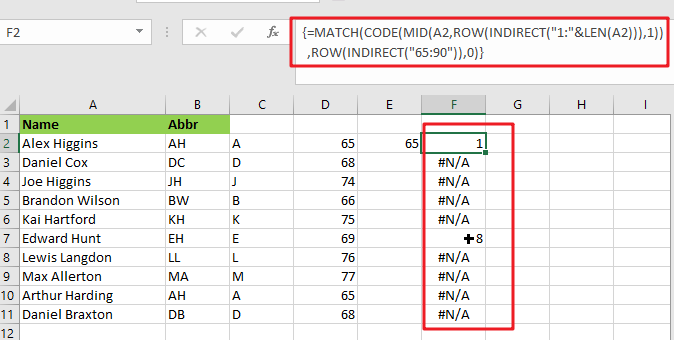The MATCH function returns either a number or the #N/A error. Because numbers represent capital letters.

`{=IF(ISNUMBER(MATCH(CODE(MID(A2,ROW(INDIRECT("1:"&LEN(A2))),1)),ROW(INDIRECT("65:90")),0)),MID(A2,ROW(INDIRECT("1:"&LEN(A2))),1),"")}`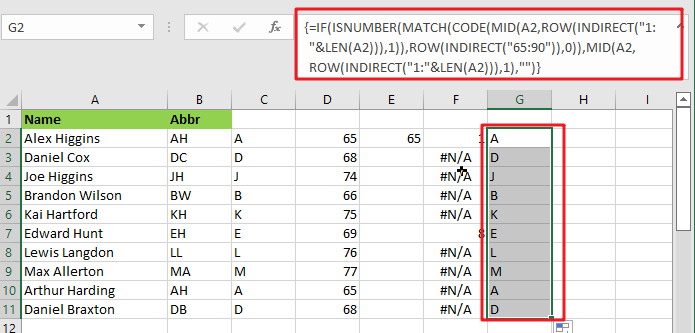The ISNUMBER function is used with the IF function to filter results. Only characters with ASCII codes between 65 and 90 will be included in the final array, reconstructed using the TEXTJOIN method to produce the final abbreviation or acronym.

## Abbreviate Names And Words In MS Excel 2016 Or Older Versions

As in Excel 2016 and earlier versions, the formula discussed above would not work, so the TRIM function is available in Excel 2016 and earlier versions, so we use it to abbreviate names and words.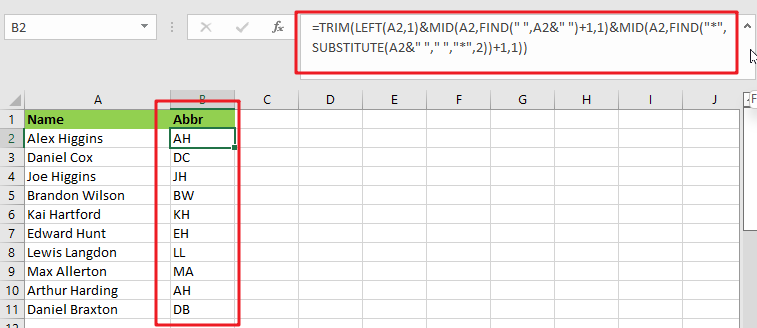### General Formula:

The formula we would use in MS Excel 2016 and earlier versions to abbreviate names and words as follows.

`=TRIM(LEFT(Text,1)&MID(Text,FIND(" ",Text&" ")+1,1)&MID(Text,FIND("*",SUBSTITUTE(Text&" ","*,"2))+1,1)`

### How Does This Formula Work?

From cell A2, if you want to extract the initials, enter this formula in cell B2.

`=TRIM(LEFT(A2,1)&MID(A2,FIND(" ",A2&" ")+1,1)&MID(A2,FIND("*",SUBSTITUTE(A2&" "," ","*",2))+1,1))`

Here the text string is the string you want to extract the first letters of each word.

When you press the Enter key, all of the first letters of each word in cell A2 are retrieved.### Explanation:

1. The TRIM function eliminates any extra spaces from the text string.
2. The LEFT(A2,1) function retrieves the first letter of the text string.
3. MID(A2,FIND(” “,A2&” “)+1,1) retrieves the initial letter of the second word separated by a space.
4. MID(A2,FIND(“*”,SUBSTITUTE(A2&” “,” “,”*,”2))+1,1) retrieves the initial letter of the third word separated by a space.

NOTE:

This formula only works when three or fewer words are in a cell. You can modify ” “ in the formula to different delimiters.

This formula extracts the initial characters in a case-insensitive manner; if you want the formula to always return in the upper case, include the UPPER function in the formula.

`=UPPER(TRIM(LEFT(A2,1)&MID(A2,FIND(" ",A2&" ")+1,1)&MID(A2,FIND("*",SUBSTITUTE(A2&" "," ","*",2))+1,1)))`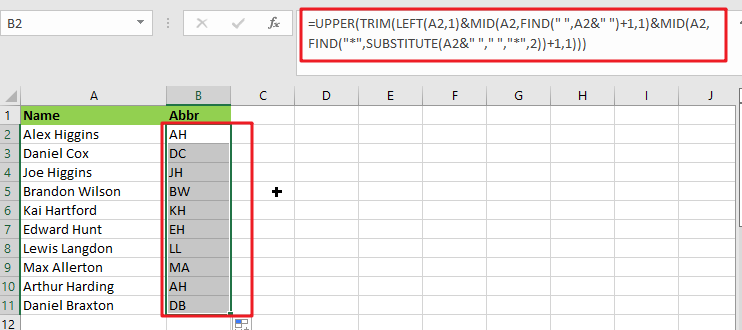### Related Functions

• Excel IF function
The Excel IF function perform a logical test to return one value if the condition is TRUE and return another value if the condition is FALSE. The IF function is a build-in function in Microsoft Excel and it is categorized as a Logical Function.The syntax of the IF function is as below:= IF (condition, [true_value], [false_value])….
• Excel TEXTJOIN function
The Excel TEXTJOIN function joins two or more text strings together and separated by a delimiter. you can select an entire range of cell references to be combined in excel 2016.The syntax of the TEXTJOIN function is as below:= TEXTJOIN  (delimiter, ignore_empty,text1,[text2])…
• Excel ROW function
The Excel ROW function returns the row number of a cell reference.The ROW function is a build-in function in Microsoft Excel and it is categorized as a Lookup and Reference Function.The syntax of the ROW function is as below:= ROW ([reference])….
• Excel MID function
The Excel MID function returns a substring from a text string at the position that you specify.The syntax of the MID function is as below:= MID (text, start_num, num_chars)…
• Excel INDIRECT function
The Excel INDIRECT function returns the cell reference based on a text string, such as: type the text string “A2” in B1 cell, it just a text string, so you can use INDIRECT function to convert text string as cell reference….
• Excel MATCH  function
The Excel MATCH function search a value in an array and returns the position of that item.The MATCH function is a build-in function in Microsoft Excel and it is categorized as a Lookup and Reference Function.The syntax of the MATCH function is as below:= MATCH  (lookup_value, lookup_array, [match_type])….
• Excel ISNUMBER function
The Excel ISNUMBER function returns TRUE if the value in a cell is a numeric value, otherwise it will return FALSE.The syntax of the ISNUMBER function is as below:= ISNUMBER (value)…
• Excel CODE function
The Excel CODE function returns the numeric ASCII value for the first character of a text string.The syntax of the CODE function is as below:= CODE  (text)…
• Excel LEN function
The Excel LEN function returns the length of a text string (the number of characters in a text string).The LEN function is a build-in function in Microsoft Excel and it is categorized as a Text Function.The syntax of the LEN function is as below:= LEN(text)…
• Excel LEFT function
The Excel LEFT function returns a substring (a specified number of the characters) from a text string, starting from the leftmost character.The LEFT function is a build-in function in Microsoft Excel and it is categorized as a Text Function.The syntax of the LEFT function is as below:= LEFT(text,[num_chars])…t)…
• Excel FIND function
The Excel FIND function returns the position of the first text string (sub string) within another text string.The syntax of the FIND function is as below:= FIND(find_text, within_text,[start_num])…
• Excel TRIM function
The Excel TRIM function removes all spaces from text string except for single spaces between words.  You can use the TRIM function to remove extra spaces between words in a string.The syntax of the TRIM function is as below:= TRIM (text)….

## Filter or Remove Columns

Assume that in MS Excel, you have a table consisting of a few columns consisting of few values, and you want to filter to remove the specified columns from the table. You might take it easy and would prefer to manually filter out to remove the desired columns from the table, without any need for the formula; then congratulations because you are thinking right.

But let me add that it would be a big deal while dealing with multiple columns containing a bulk of data in the table, and then doing this bulky task manually would be a foolish decision.

But there isn’t any need to worry about it because after carefully reading this article, filtering out to remove the desired columns will be a piece of cake for you.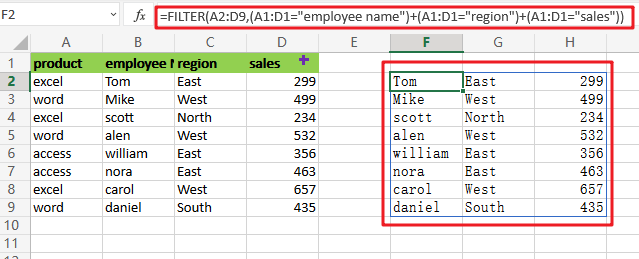So let’s get straight into it!

## General Formula

To filter out desired columns from the table, we would use the FILTER function and supply the horizontal array; the general formula is as follows:

`=FILTER(total_data,(range_name1=value1)+( range_name2=value2))`

As we have altered the above formula according to the example which we would discuss in this article to understand that how this formula works and how to use this formula:

`=FILTER(A2:D9,(A1:D1="employee name")+(A1:D1="region")+(A1:D1="sales"))`The end result is a filtered collection of data that only includes columns B, C, and D from the original data.

## Let’s See How This Formula Works:

Although FILTER is usually used to filter rows, it may also filter columns; the secret is to give an array with the same number of columns as the original data. In this example, we use boolean logic, commonly known as Boolean algebra, to create the necessary array.

Multiplication corresponds to AND logic in Boolean algebra and addition corresponds to OR logic. In the following example, we use Boolean algebra using OR logic (addition) to target only the columns “employee name”, “region”, and “sales” as follows:

After evaluating each expression, we obtain three arrays of TRUE/FALSE values:

`=(A1:D1="employee name")`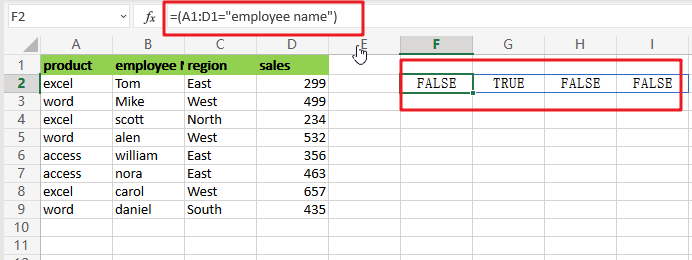`=(A1:D1="employee name")+(A1:D1="region")+(A1:D1="sales")`

The arithmetic action (addition) turns TRUE and FALSE values to 1s and 0s, so think of it like this: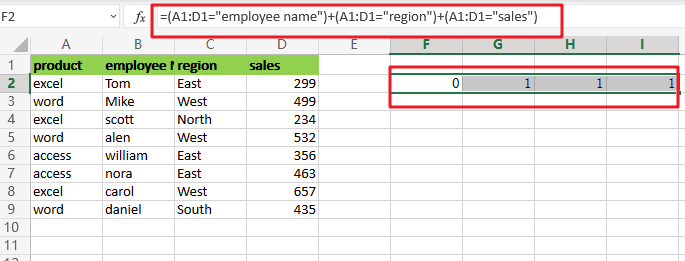This is sent straight to the FILTER function as the include argument:

`=FILTER(A2:D9,{0,1,1,1})`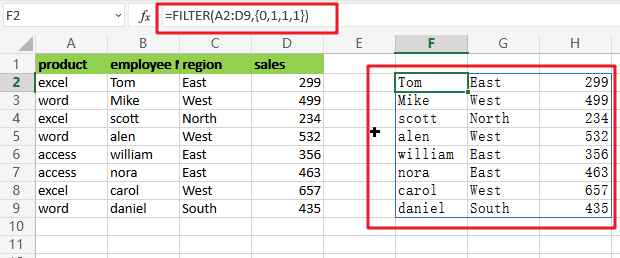In the source data, there are 4 columns and 4 values in the array, all of which are either 1 or 0. FILTER utilizes this array as a filter to include columns 2, 3, and 4 from the given data. Column  1 has been eliminated. In other words, the only columns that remain are those linked with 1s.

## Removing Columns Using the MATCH and Filter Functions

Applying OR logic with addition as demonstrated above works OK, but it does not scale well and makes it hard to utilize a range of numbers from a worksheet as the criterion. To create the include argument more quickly, you may use the MATCH function in conjunction with the ISNUMBER function as seen below:

`=FILTER(A2:D9,ISNUMBER(MATCH(A1:D1,{"employee name","region","sales"},0)))`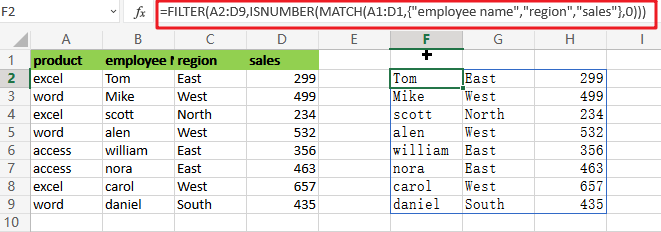`=MATCH(A1:D1,{"employee name","region","sales"},0)`

The MATCH function is set to seek for all column headings in the array constants ,”employee name“,”region” and “sales” as illustrated. We implement it this way so that the MATCH result has dimensions consistent with the original data, which has 4 columns. Also, the third parameter in MATCH is set to 0 to force an exact match.

MATCH provides an array that looks like this when it runs: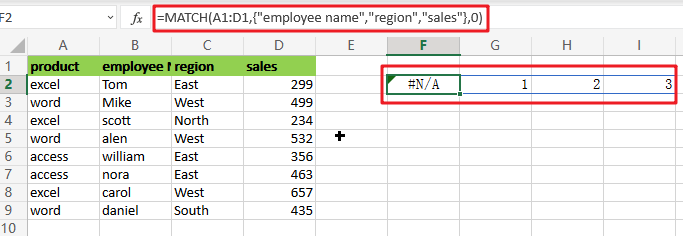`=ISNUMBER(MATCH(A1:D1,{"employee name","region","sales"},0))`

This array is sent straight to ISNUMBER, which produces another array: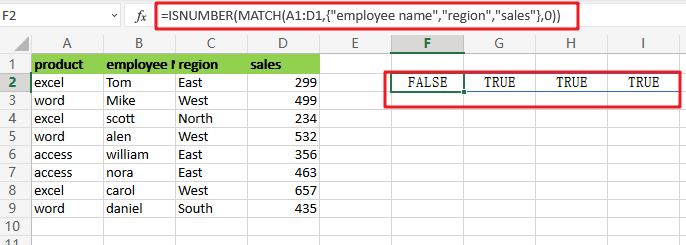Like the one above, this array is horizontal and has 4 values separated by commas. Column 1 is removed using the array by FILTER.

### Related Functions

• Excel ISNUMBER function
The Excel ISNUMBER function returns TRUE if the value in a cell is a numeric value, otherwise it will return FALSE.The syntax of the ISNUMBER function is as below:= ISNUMBER (value)…
• Excel Filter function
The FILTER function extracts matched records from a collection of data using one or more logical checks. The include argument specifies logical tests, which might encompass a wide variety of formula conditions.==FILTER(array,include,[if empty])…
• Excel MATCH  function
The Excel MATCH function search a value in an array and returns the position of that item.The MATCH function is a build-in function in Microsoft Excel and it is categorized as a Lookup and Reference Function.The syntax of the MATCH function is as below:= MATCH  (lookup_value, lookup_array, [match_type])….

## Filter or Extract for Multiple OR Criteria

If you are an valid MS Excel user, you have probably come across a situation where you wanted to filter the data in a separate table with specific criteria. You could do this task manually, which is also acceptable when dealing with a few data items. But if you got an assignment to filter out multiple items from a table consisting of a lot of data along with certain criteria, then doing these kinds of tasks manually would definitely be a stupid decision because this would not only waste your precious time, but you would also get tired of it and won’t complete your task on time.

But don’t be worry about it; for getting out of this fix and filtering out multiple data with specific criteria, all you have to do is read this article carefully.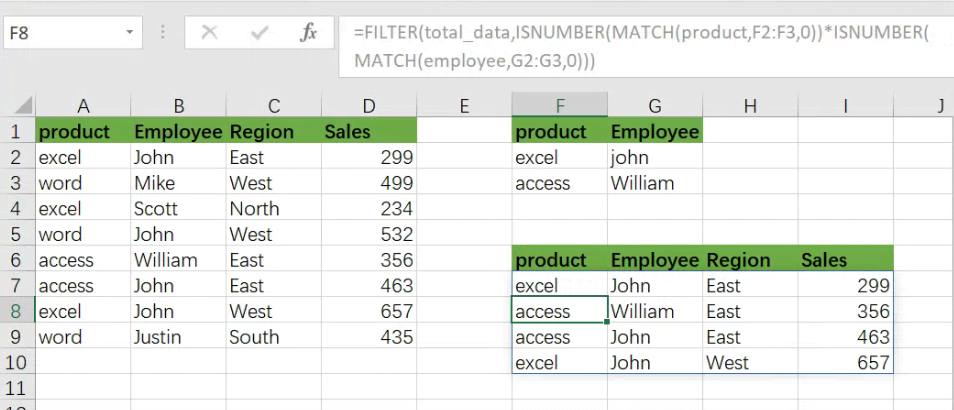So let’s dive into it.

## General formula:

The formula below would help you filter out multiple data with specific criteria within a few seconds.

As we have altered the following formula according to the example which we would discuss in this article to understand that how this formula works and how to use this formula:

` =FILTER(total_data,ISNUMBER(MATCH(product,F2:F3,0))*ISNUMBER(MATCH(employee,G2:G3,0)))`In the formula stated above, we are using the filter function along with the Match function, where ranges are specified for products(A2:A9), employee (B2:B9), and regions (C2:C9).

This formula produces information when the product is “excel” or “access”, AND the employee are “john” or “William”.

## Syntax Explanation:

Before we dive into the formula for getting the job done effectively, we need to understand each syntax so that we can know how each syntax helps to Filter with multiple OR criteria :

• `Filter`: This tool helps to narrow down or filter out a variety of data depending on user-defined criteria.
• `Comma symbol` (,): In Excel, this symbol functions as a separator and plays a vital role in separating a list of values.
• `Parenthesis` (): Its primary role is to group and separate elements.
• `ISNUMBER`: The ISNUMBER function determines if a value in a cell or a value derived from another formula is a number. ISNUMBER returns either “`true`” or “`false`.”
• `MATCH`: The MATCH function looks for a given item in a range of cells and returns the item’s relative location in the range.

## Let’s See How This Formula Works:

Criteria for filtering out multiple data are entered in the range F2:G3 in this example. The formula’s rationale is as follows: the product is “excel” or “access”, AND the employee are “john” or “William”.

This formula’s filtering logic (the include parameter) is used with the ISNUMBER and MATCH functions and boolean logic in an array operation.

MATCH is set up “backward,” using lookup values from the data and criteria for the lookup array. For example, the first requirement is that the product be either “excel” or “access”. MATCH is configured as follows to apply this condition:

`=MATCH(product,F2:F3,0) // look for excel product`

As in the example, there are 8 values in the data; that’s why we get an array with 8 values that looks like the following: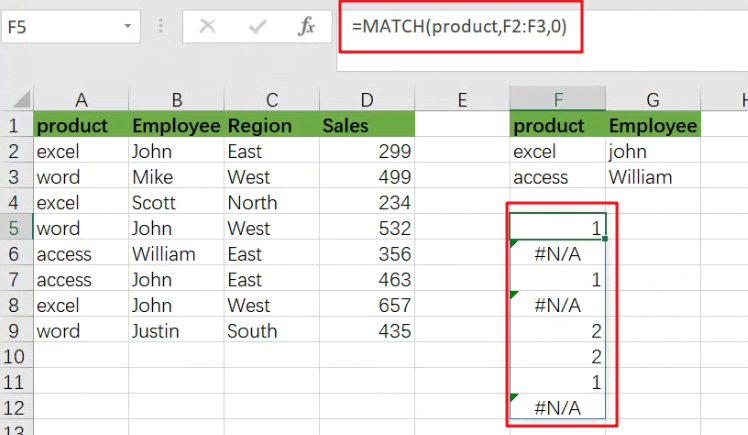The above array would include `#N/A` errors (no match) or numbers (match). The numbers on the notice refer to either “excel” or “access” products. To turn this array into TRUE and FALSE values, the MATCH function is wrapped in the ISNUMBER function:

`=ISNUMBER(MATCH(product,F2:F3,0))`

which results in an array like the following one: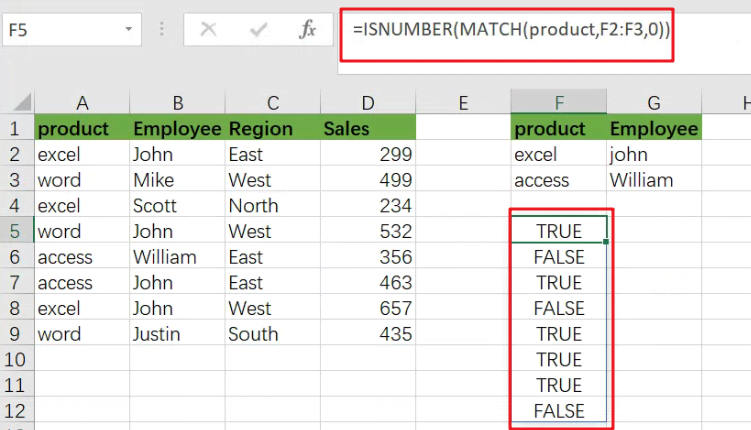TRUE values in this array match a “excel” or “access”.

The exclusive formula has two expressions similar to the ones used for the FILTER function’s include argument.

Following the evaluation of MATCH and ISNUMBER, we get two arrays containing TRUE and FALSE values. The arithmetic action of multiplying these arrays together converts the TRUE and FALSE values to 1s and 0s.

Following the laws of boolean arithmetic, the outcome is a single array which is stated as follows: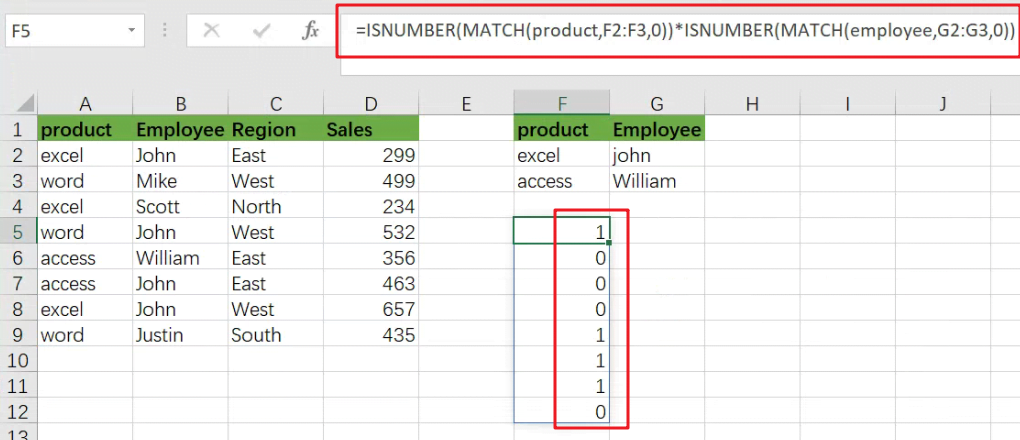which is sent as an argument to the FILTER function like the following:

`=FILTER(B5:D16,{1;0;0;0;0;1;0;0;0;0;0;1})`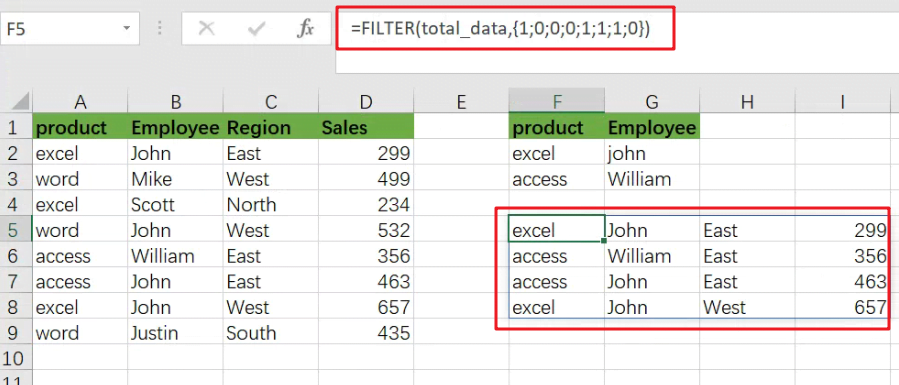### Related Functions

• Excel ISNUMBER function
The Excel ISNUMBER function returns TRUE if the value in a cell is a numeric value, otherwise it will return FALSE.The syntax of the ISNUMBER function is as below:= ISNUMBER (value)…
• Excel Filter function
The FILTER function extracts matched records from a collection of data using one or more logical checks. The include argument specifies logical tests, which might encompass a wide variety of formula conditions.==FILTER(array,include,[if empty])…
• Excel MATCH  function
The Excel MATCH function search a value in an array and returns the position of that item.The MATCH function is a build-in function in Microsoft Excel and it is categorized as a Lookup and Reference Function.The syntax of the MATCH function is as below:= MATCH  (lookup_value, lookup_array, [match_type])….